# RD Sharma Solutions for Class 11 Maths Chapter 24 The Circle

## RD Sharma Solutions Class 11 Maths Chapter 24 – Avail Free PDF Updated for 2021 – 22

RD Sharma Solutions for Class 11 Maths Chapter 24 – The Circle are provided here for students to score good marks in the board exams. In this section, we shall study about the circle and also find the equation of any circle whose center and radius are given. Experts at BYJU’S have formulated the RD Sharma Class 11 Solutions for Maths in the most lucid and easy manner based on the latest CBSE syllabus and guidelines provided by the CBSE board.

The RD Sharma Solutions are prepared using shortcut techniques to help students grasp the concepts faster and to make learning fun. Students who find difficulty in understanding the concepts can refer to RD Sharma Class 11 Maths Solutions, which is the best reference material. The solutions to this chapter are available in the pdf format, which can be downloaded easily from the links provided below.

Chapter 24 – The Circle contains three exercises and the RD Sharma Solutions present in this page provide solutions to the questions present in each exercise. Now, let us have a look at the concepts discussed in this chapter.

• Standard equation of a circle.
• Some particular cases.
• General equations of a circle.
• Diameter form of a circle.

## Download the pdf of RD Sharma Solutions for Class 11 Maths Chapter 24 – The Circle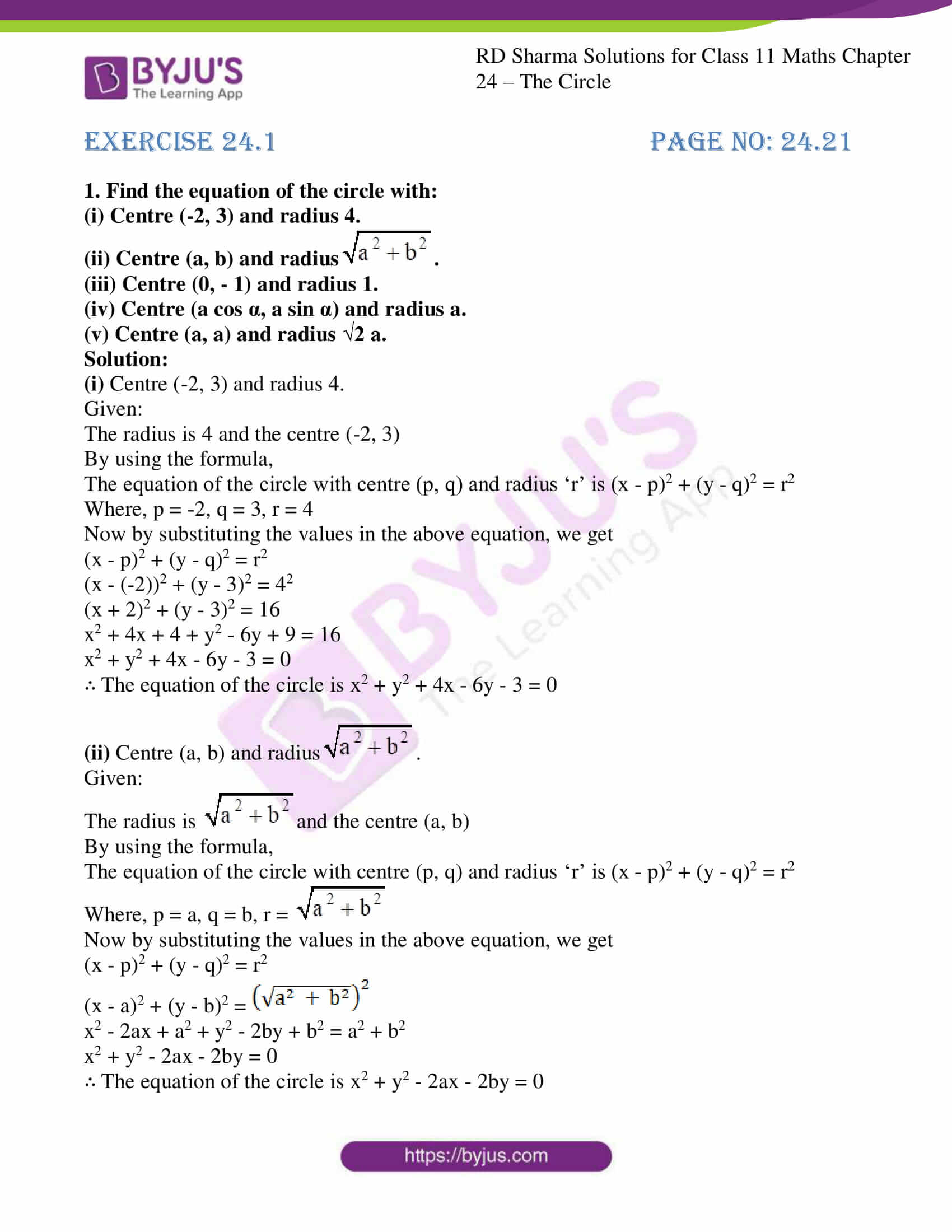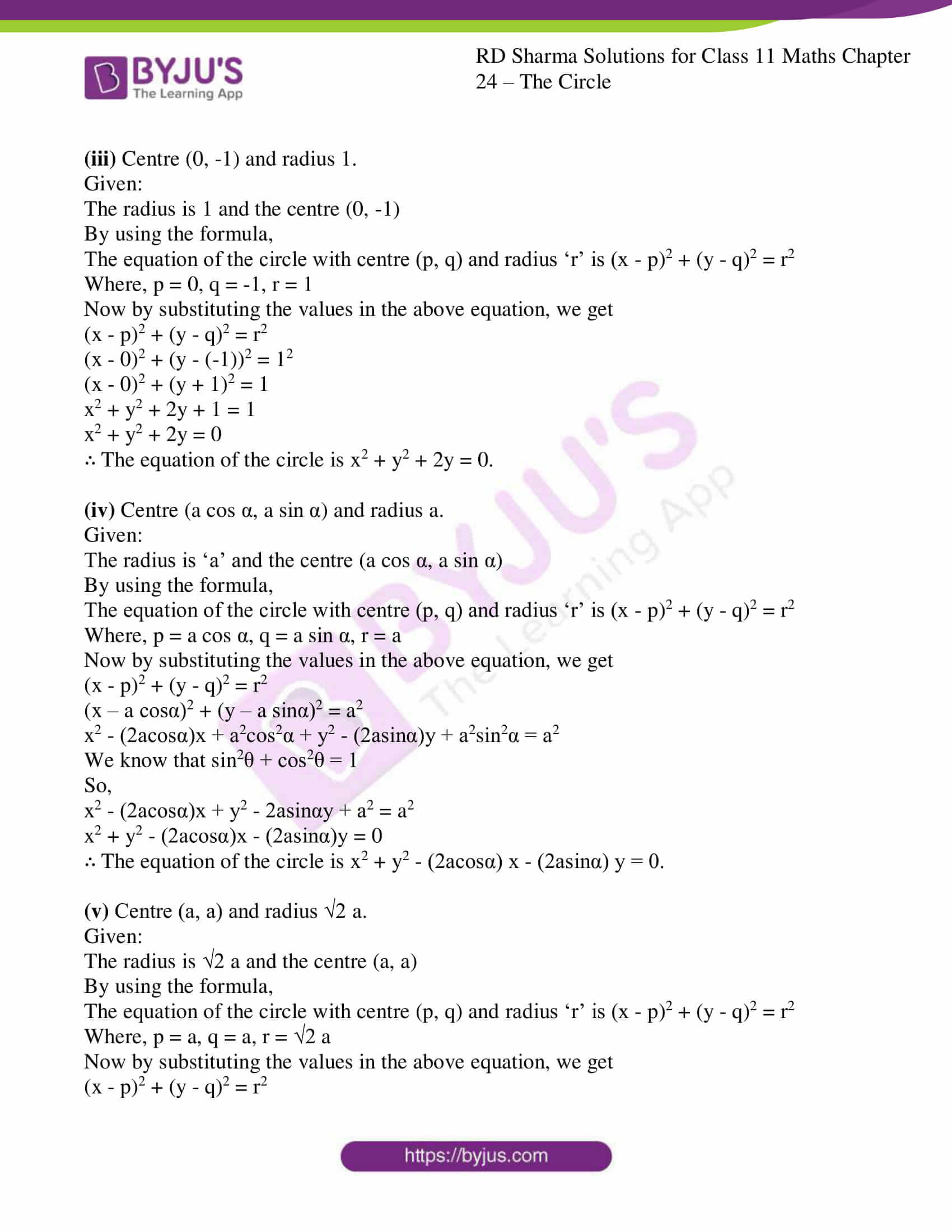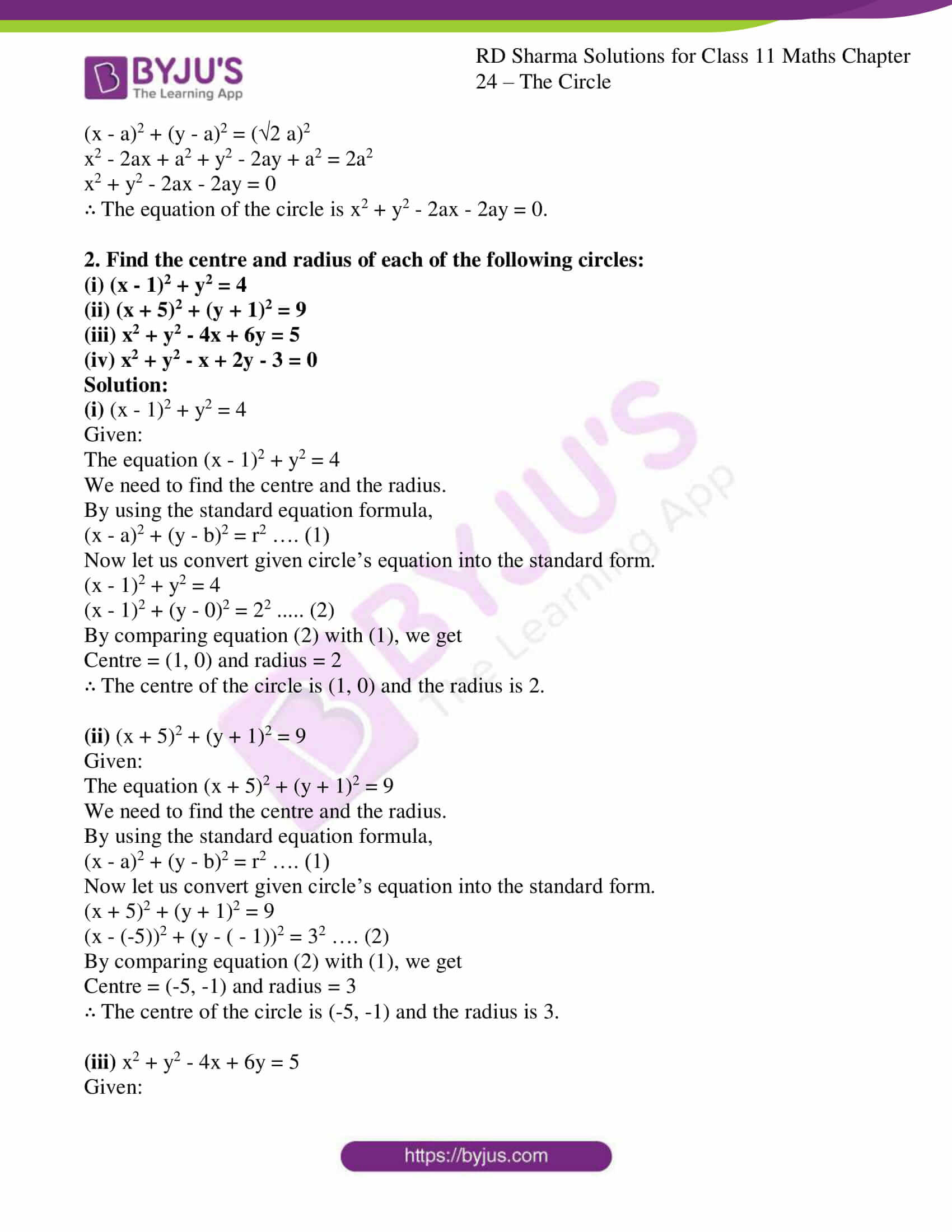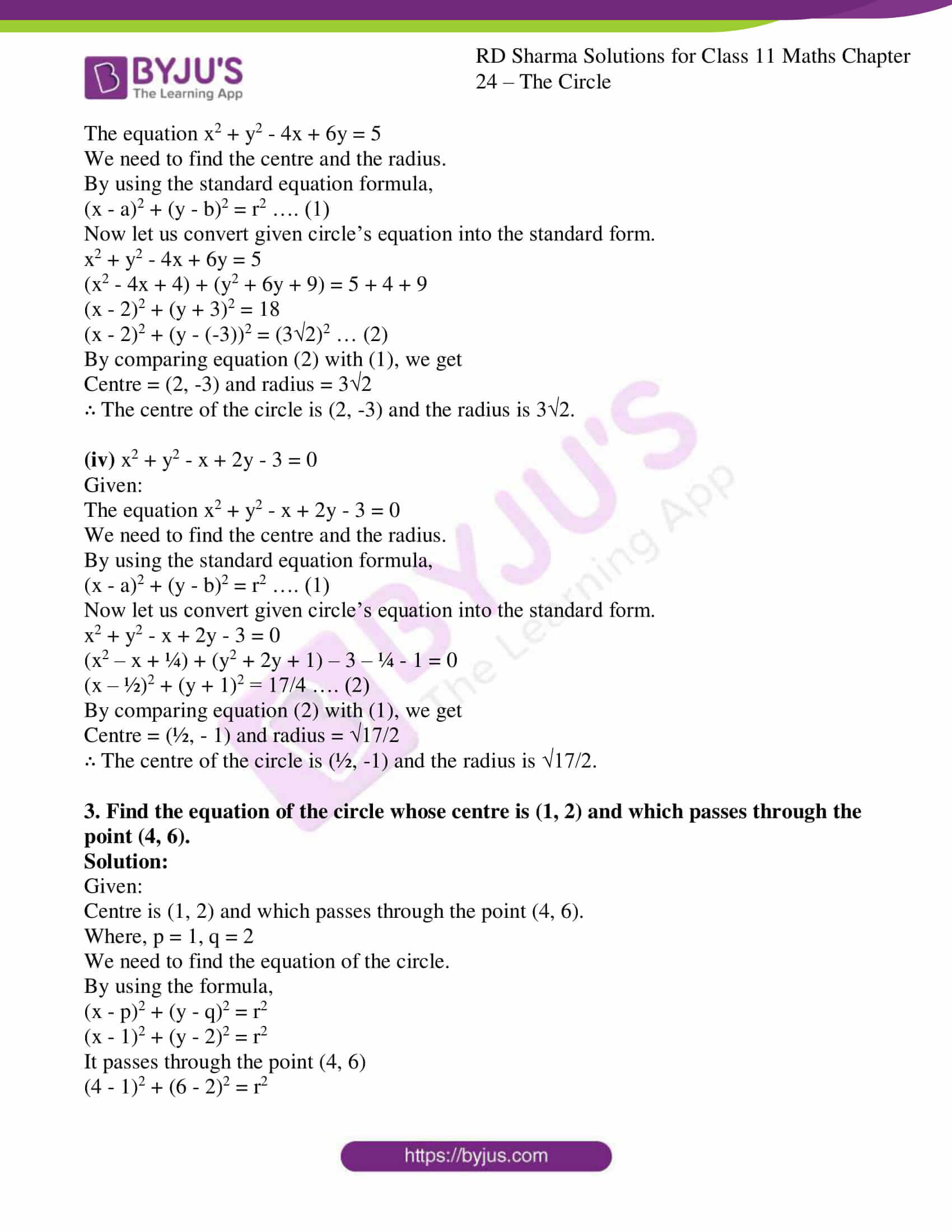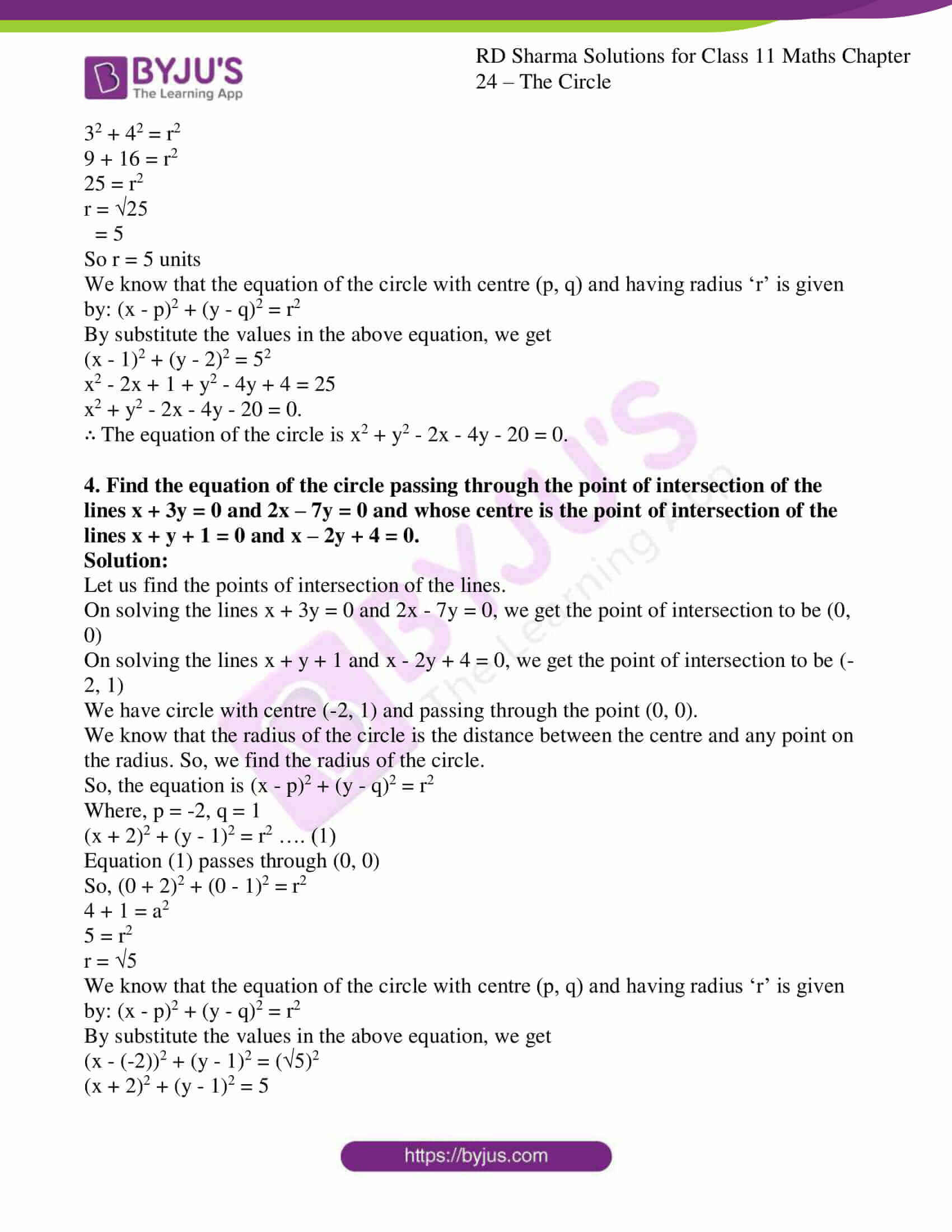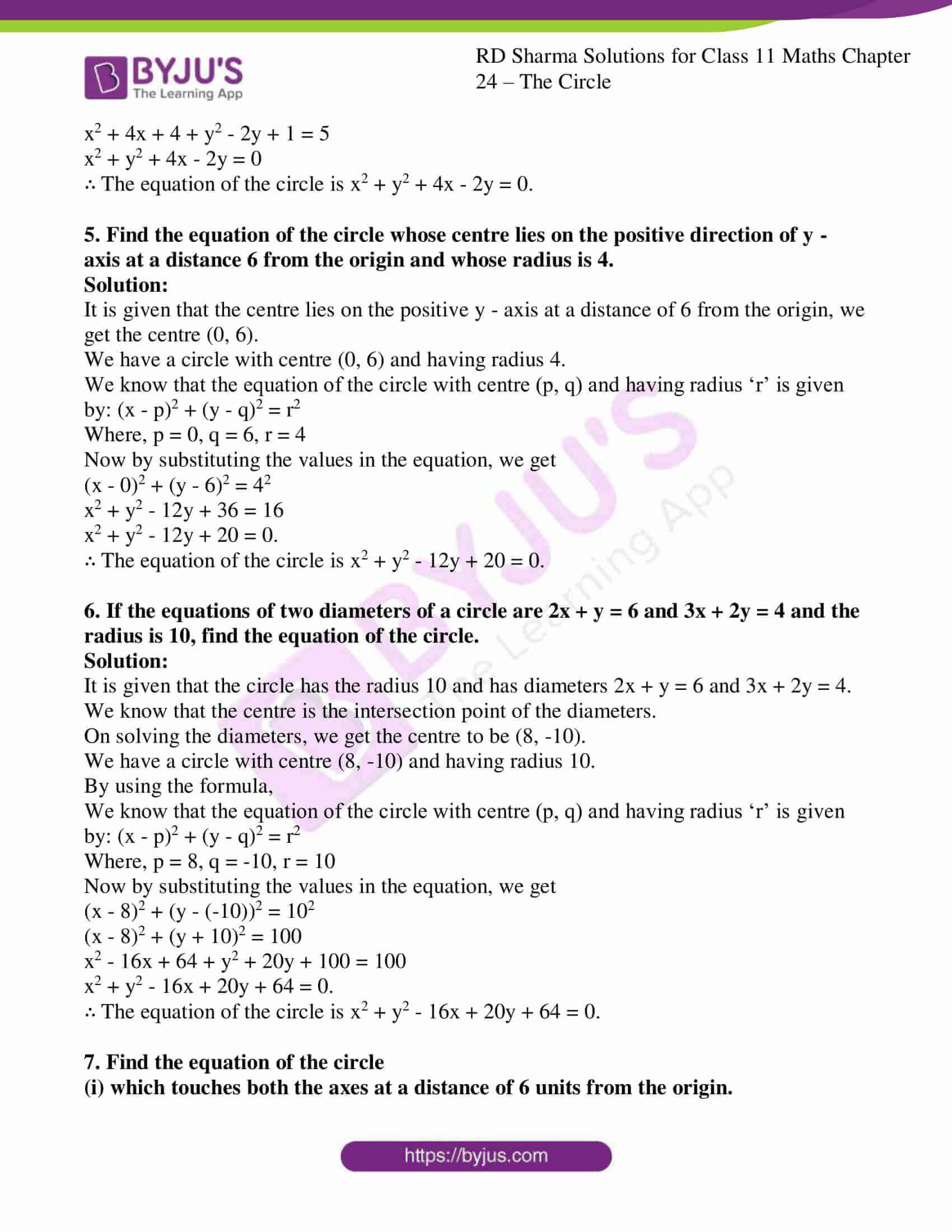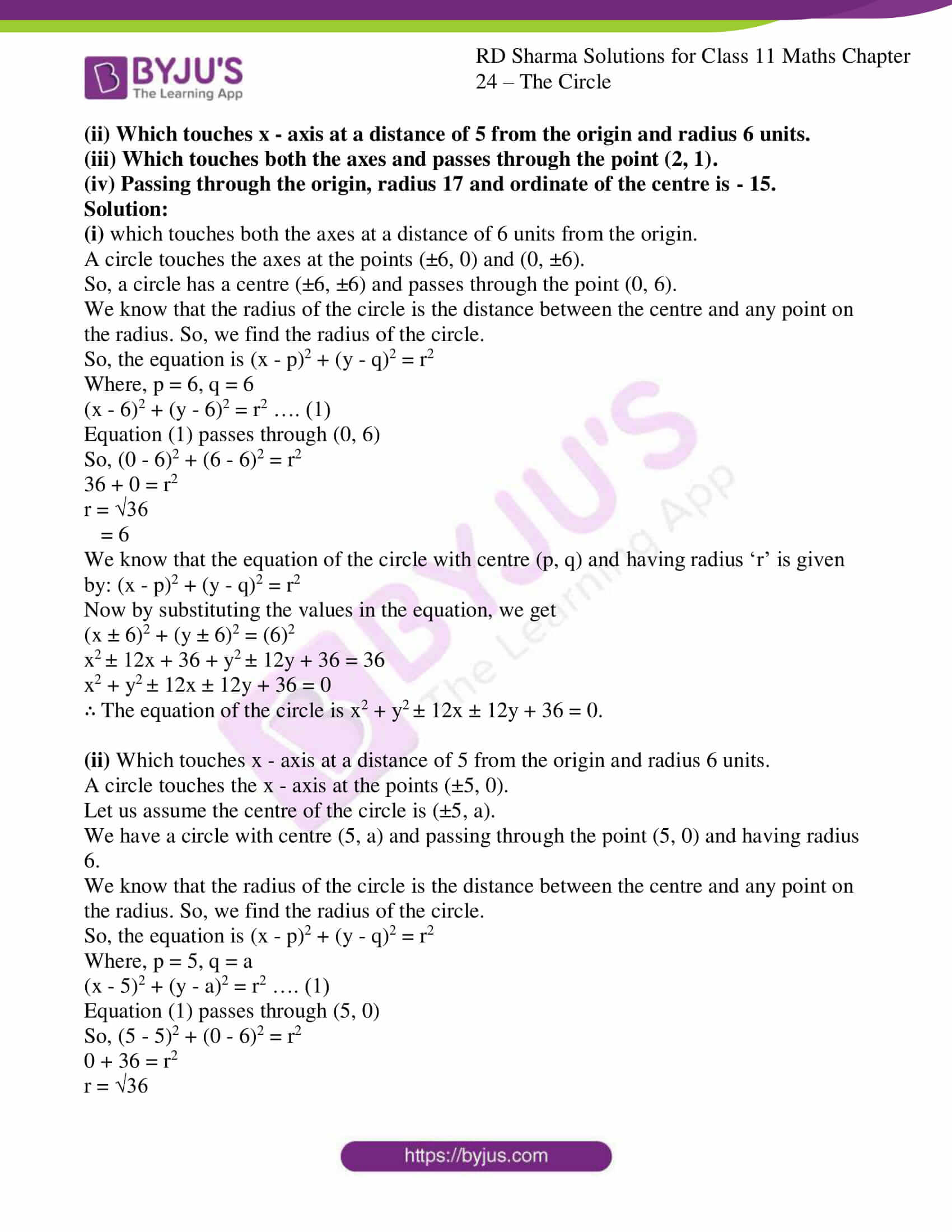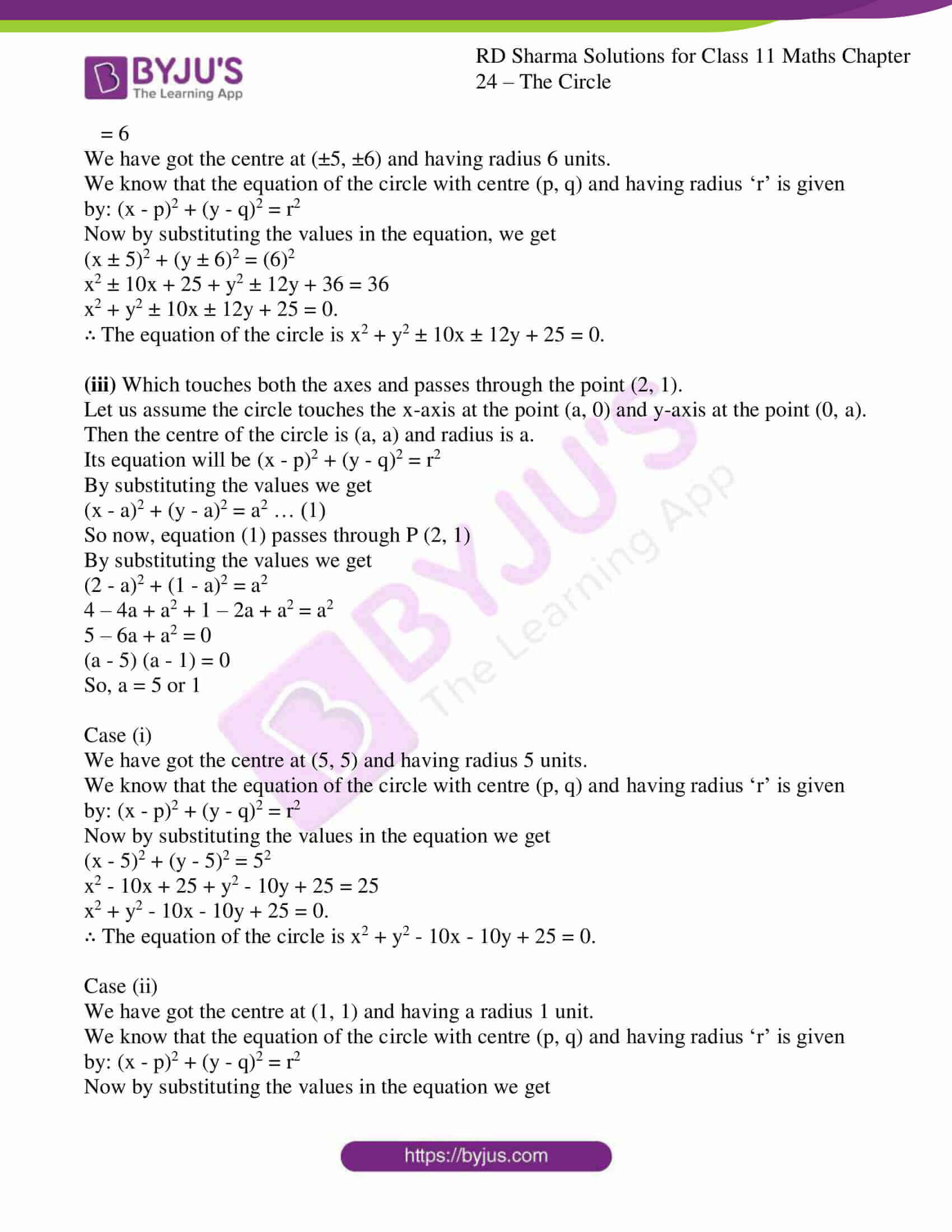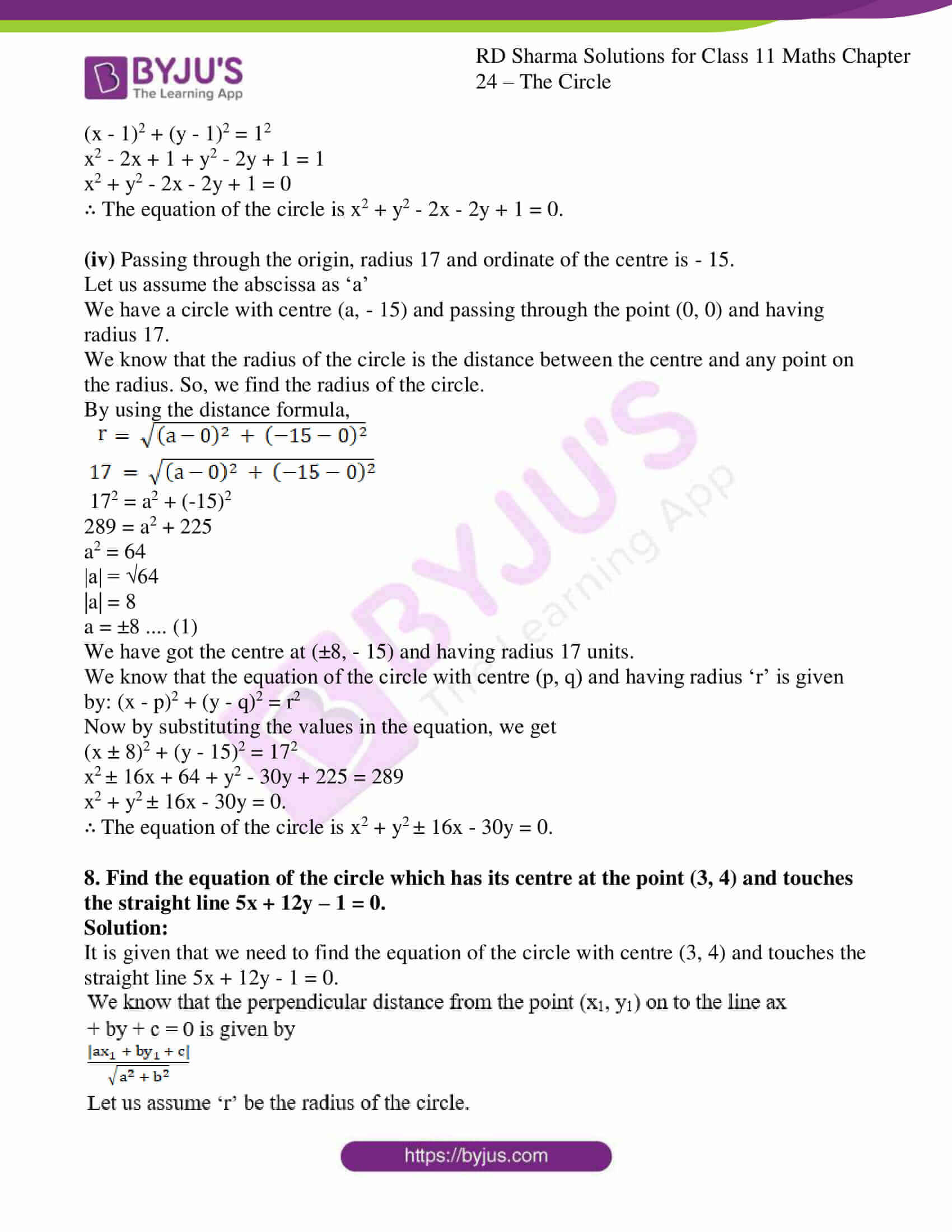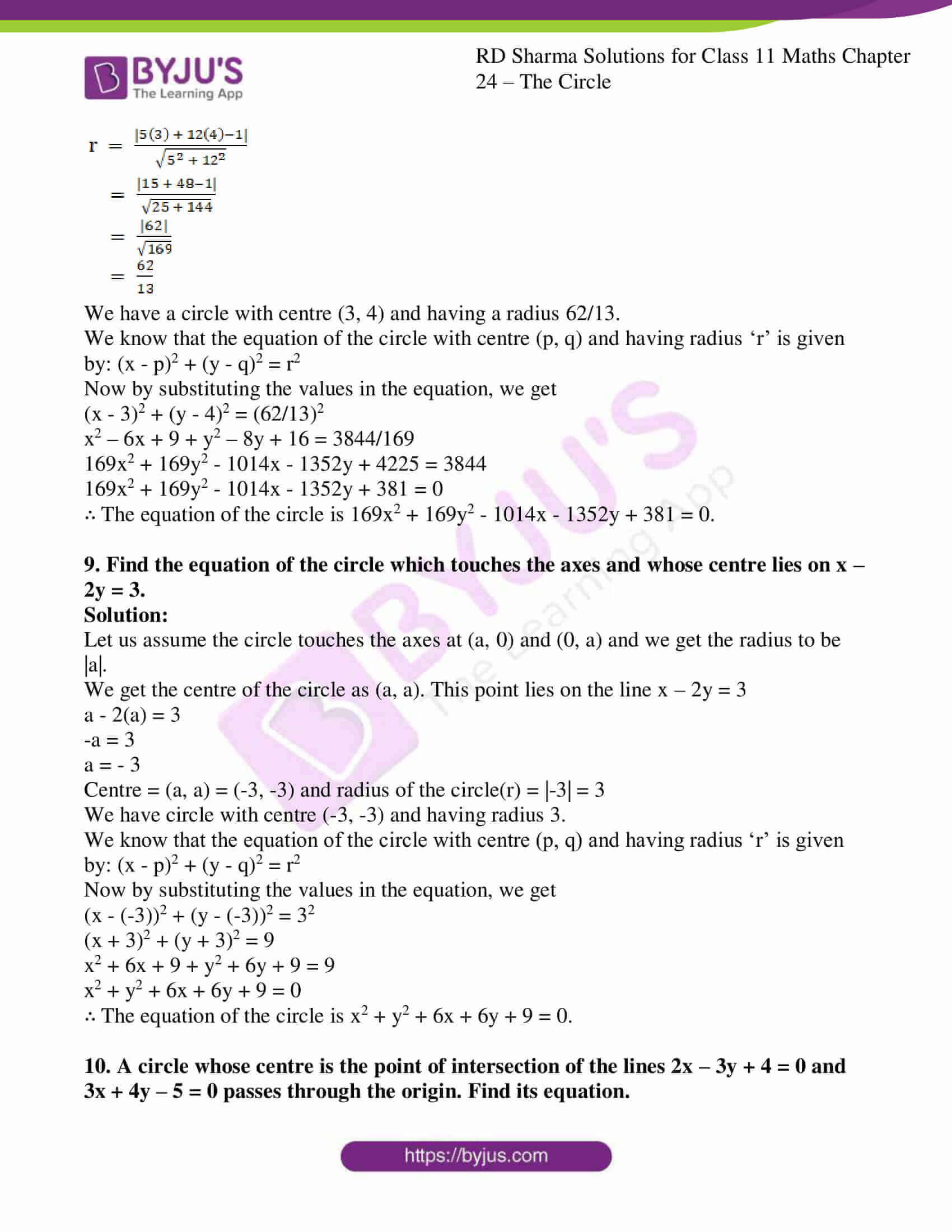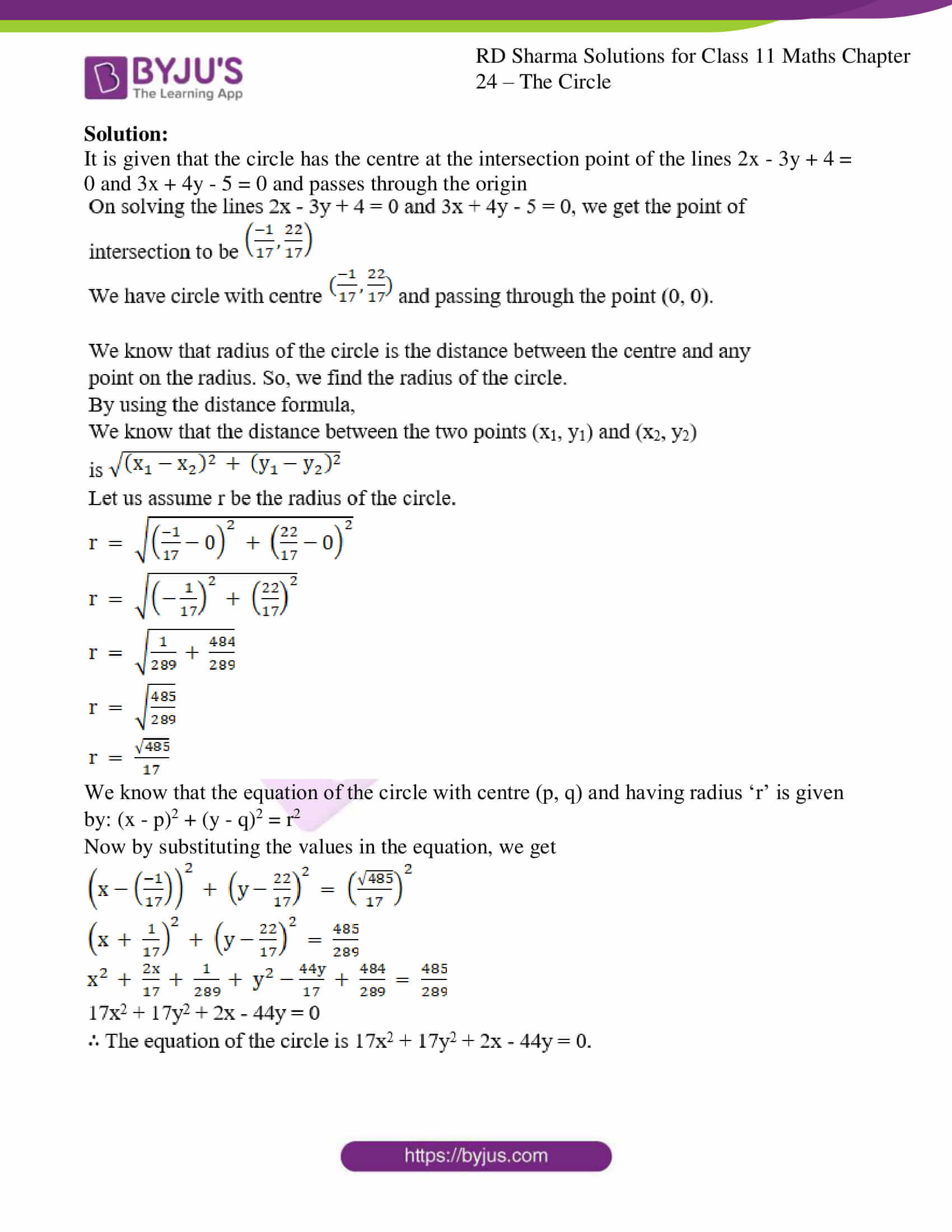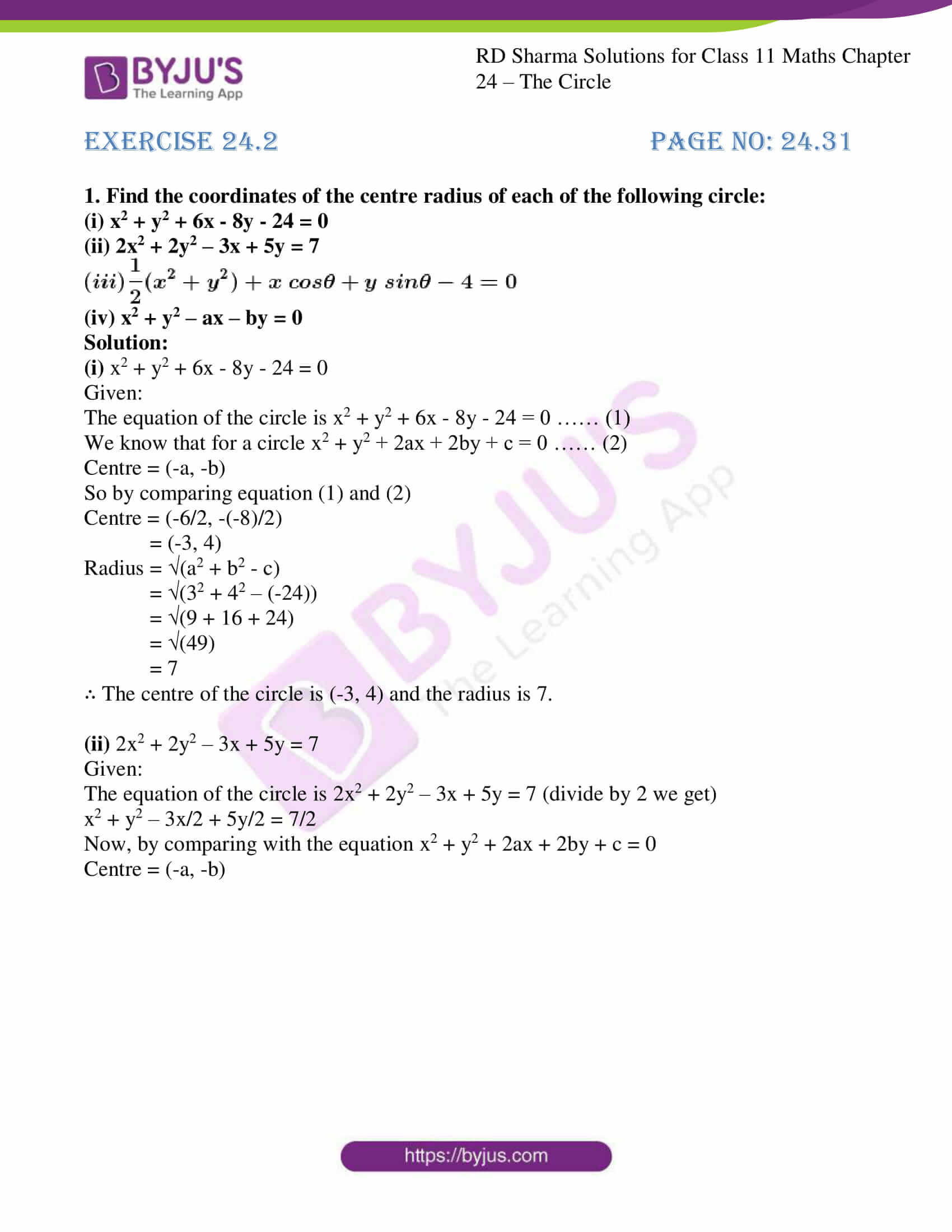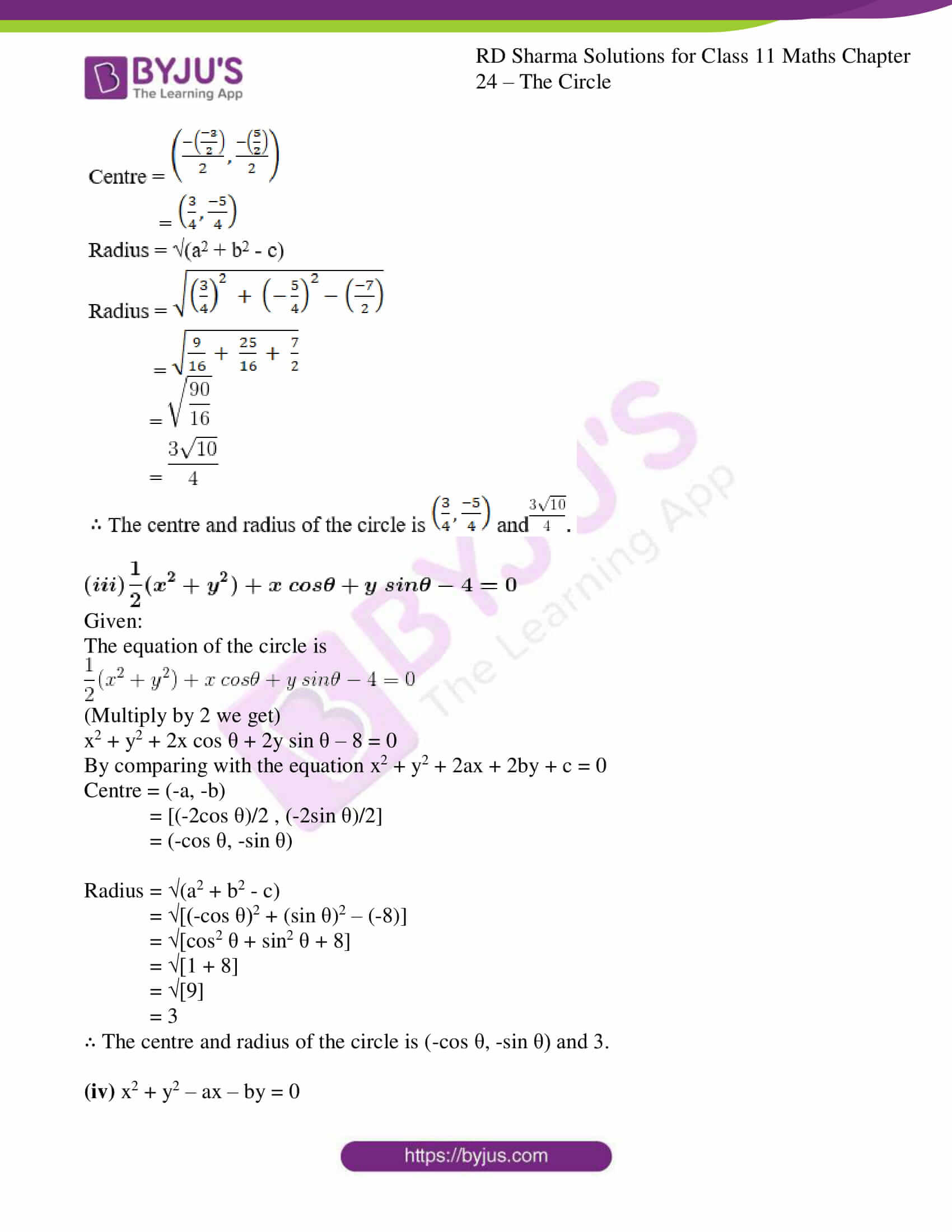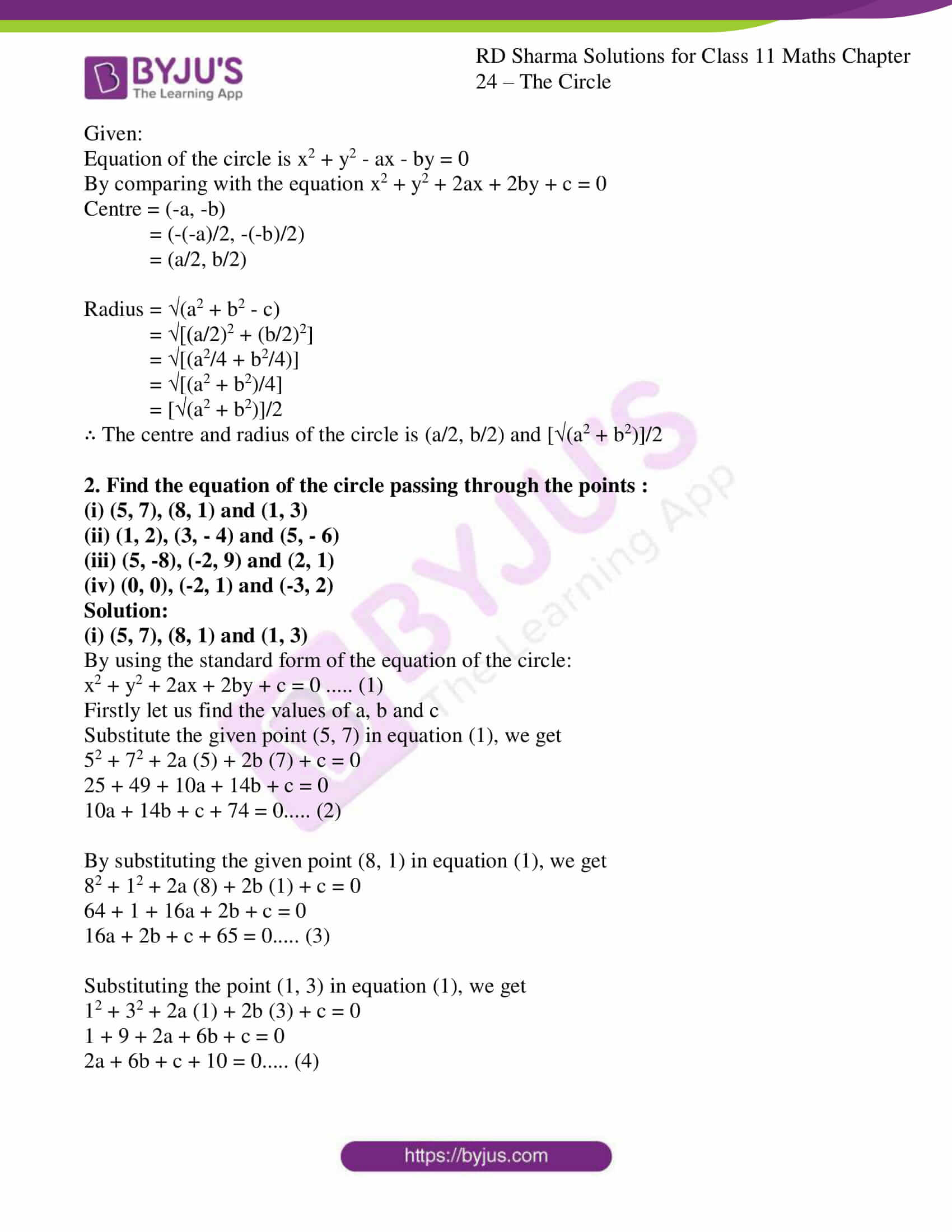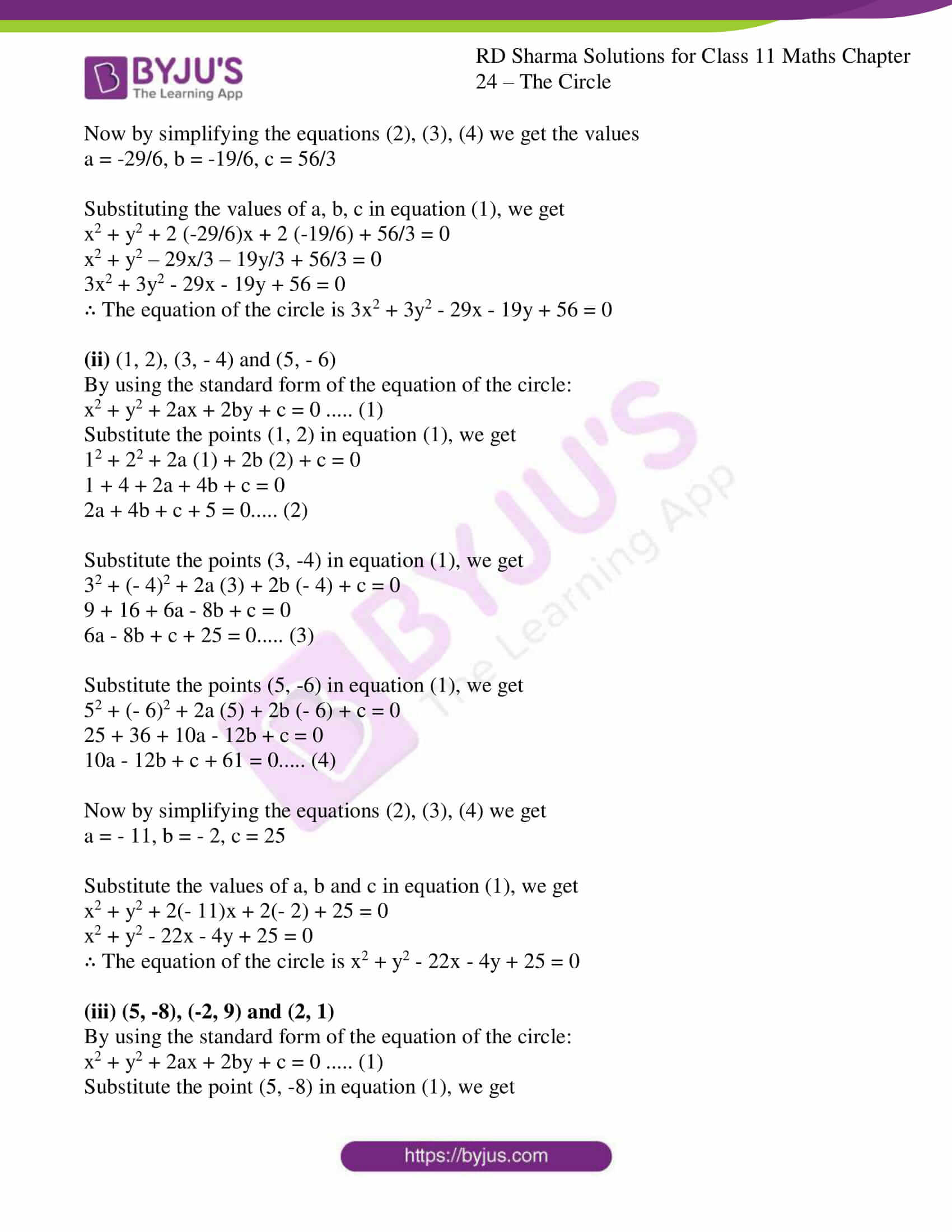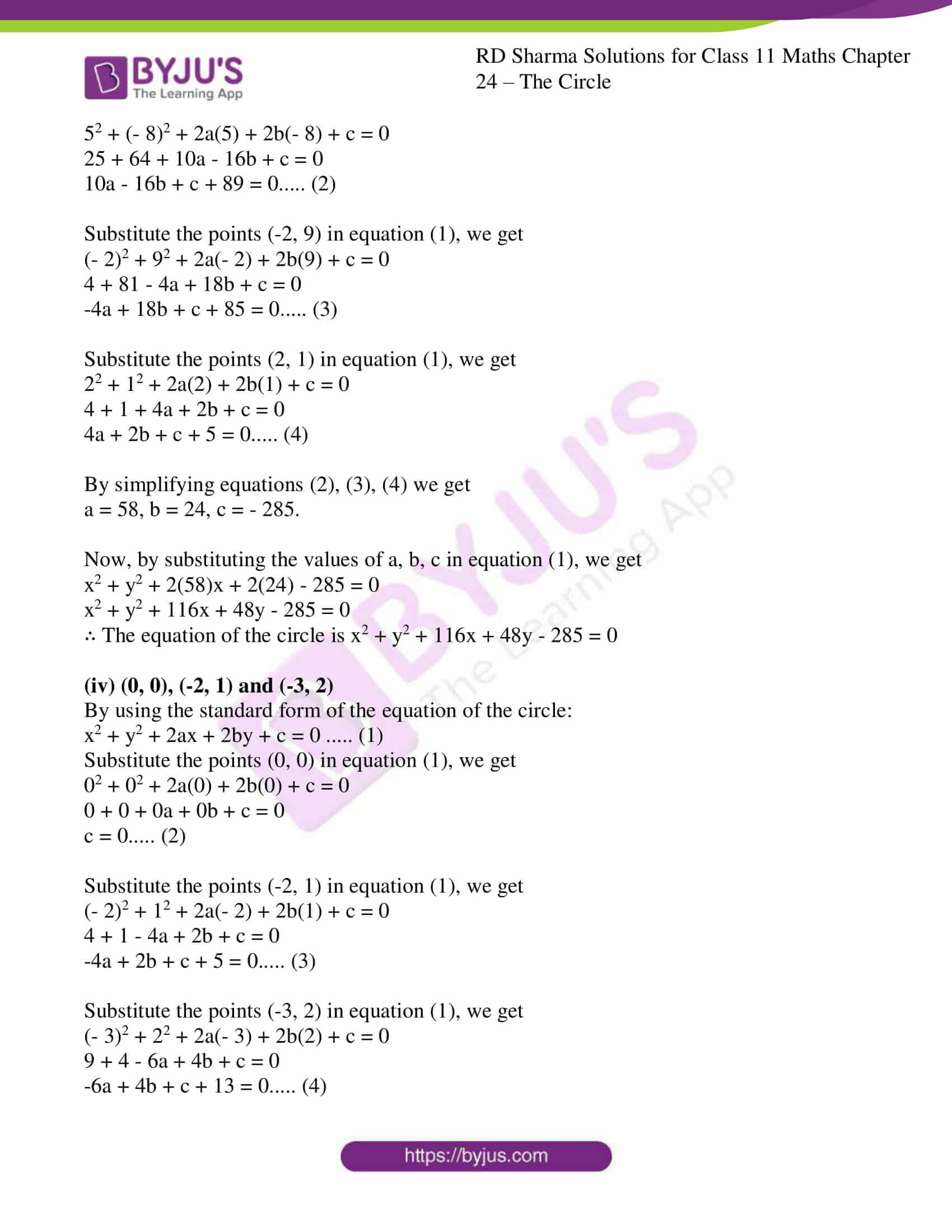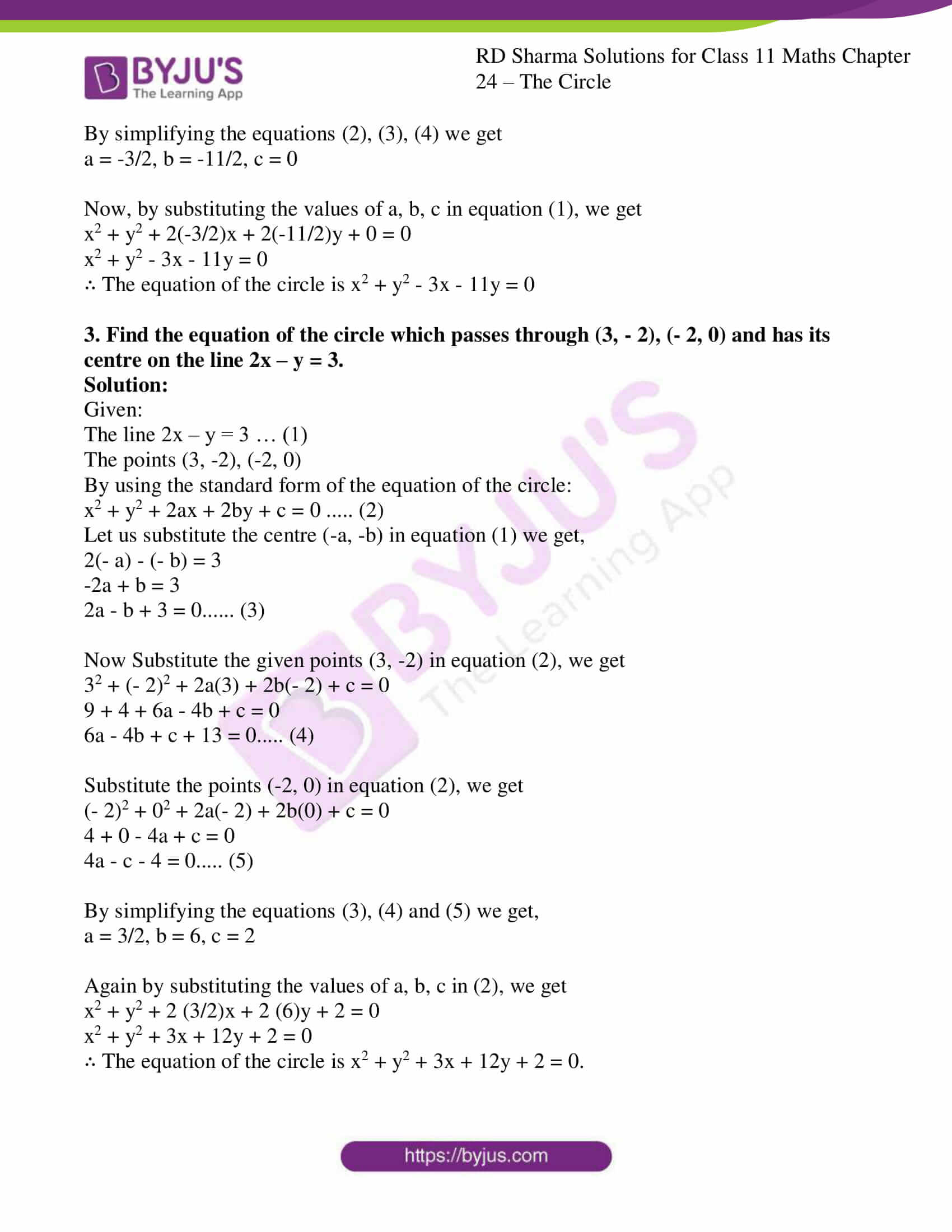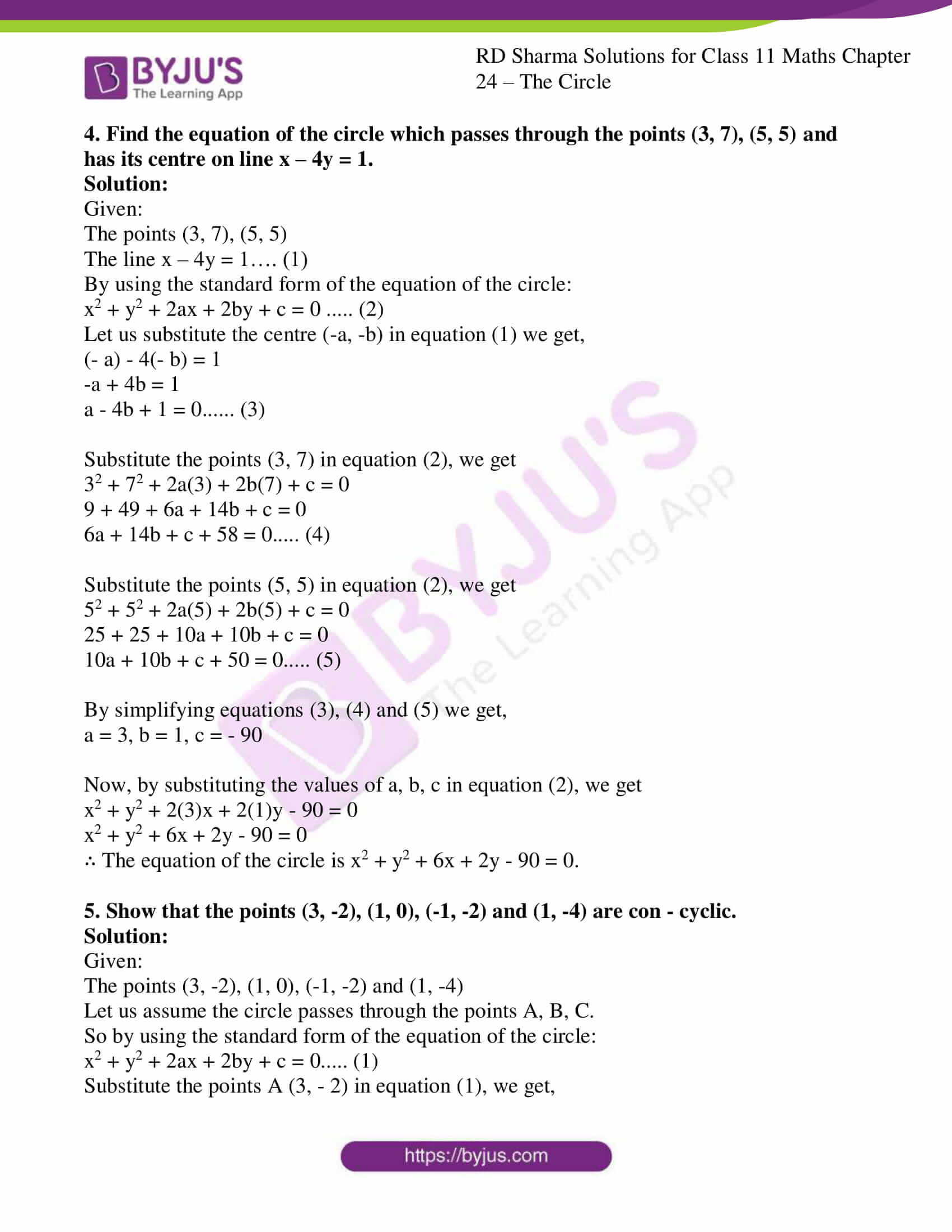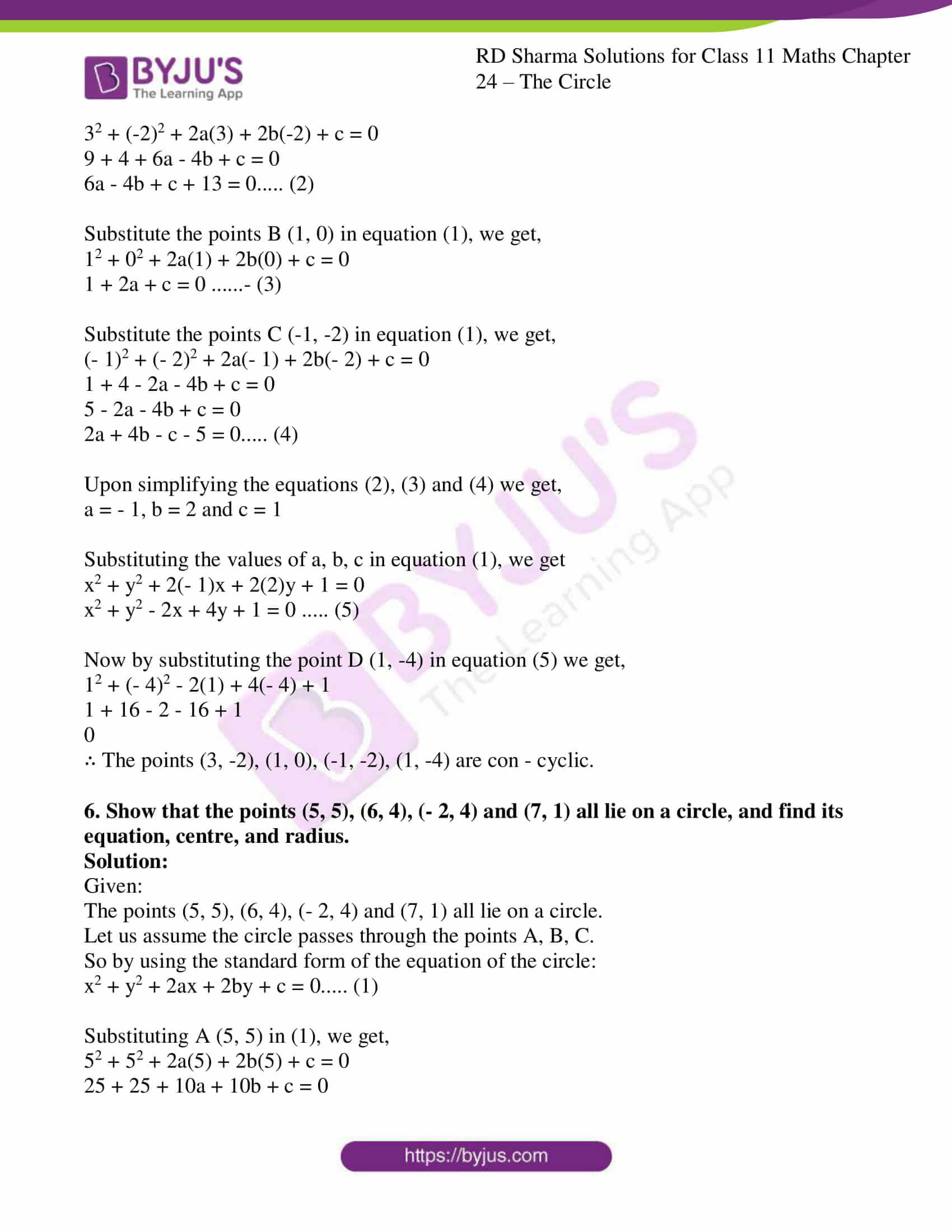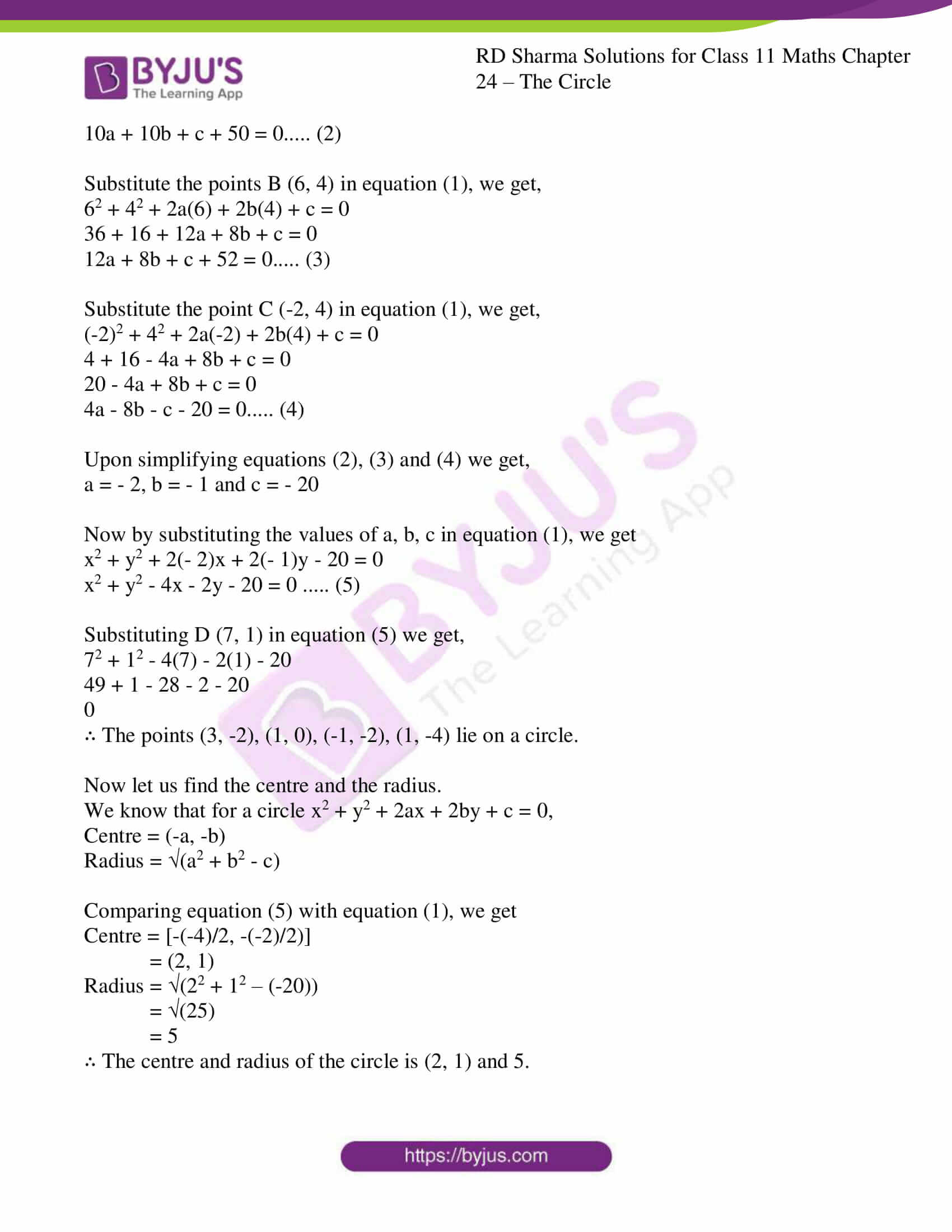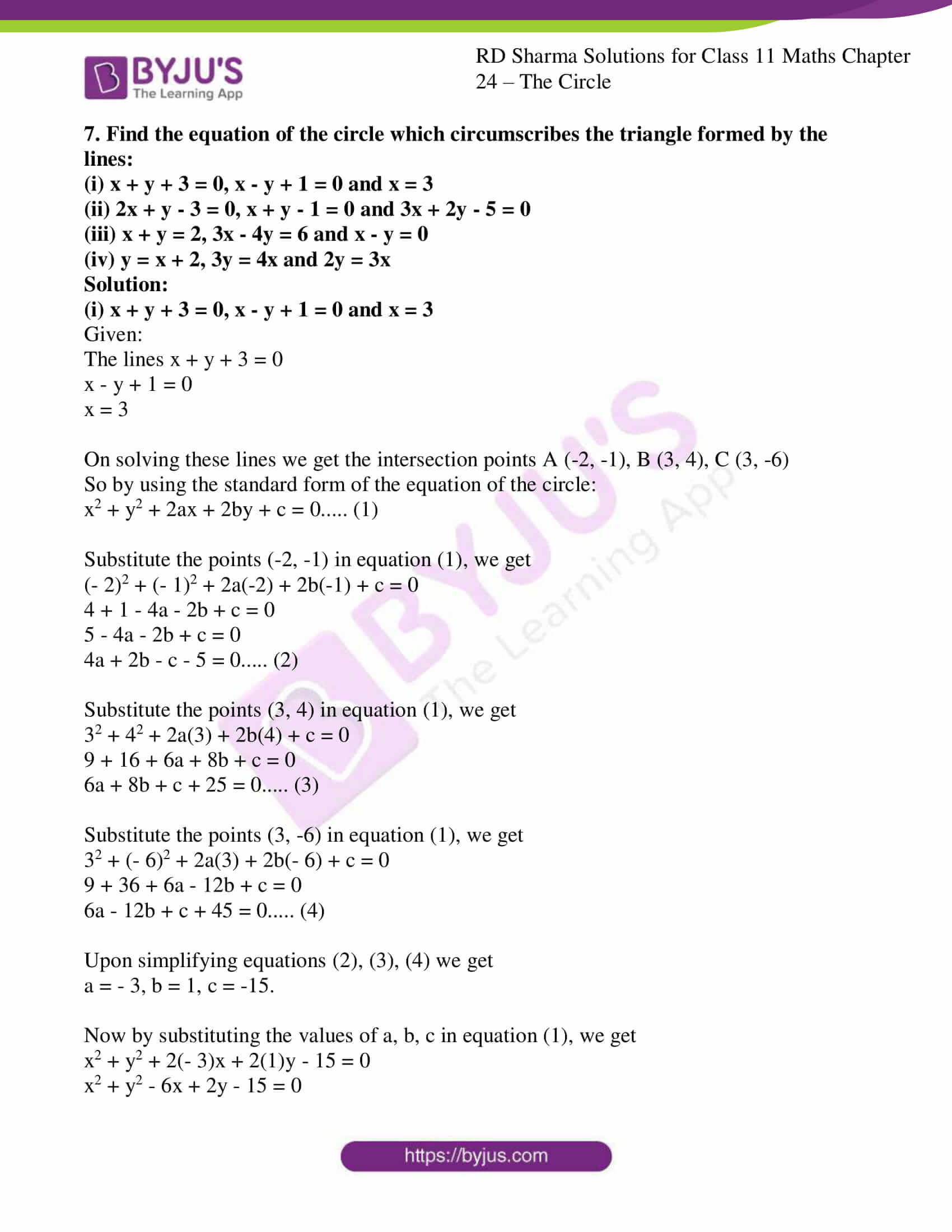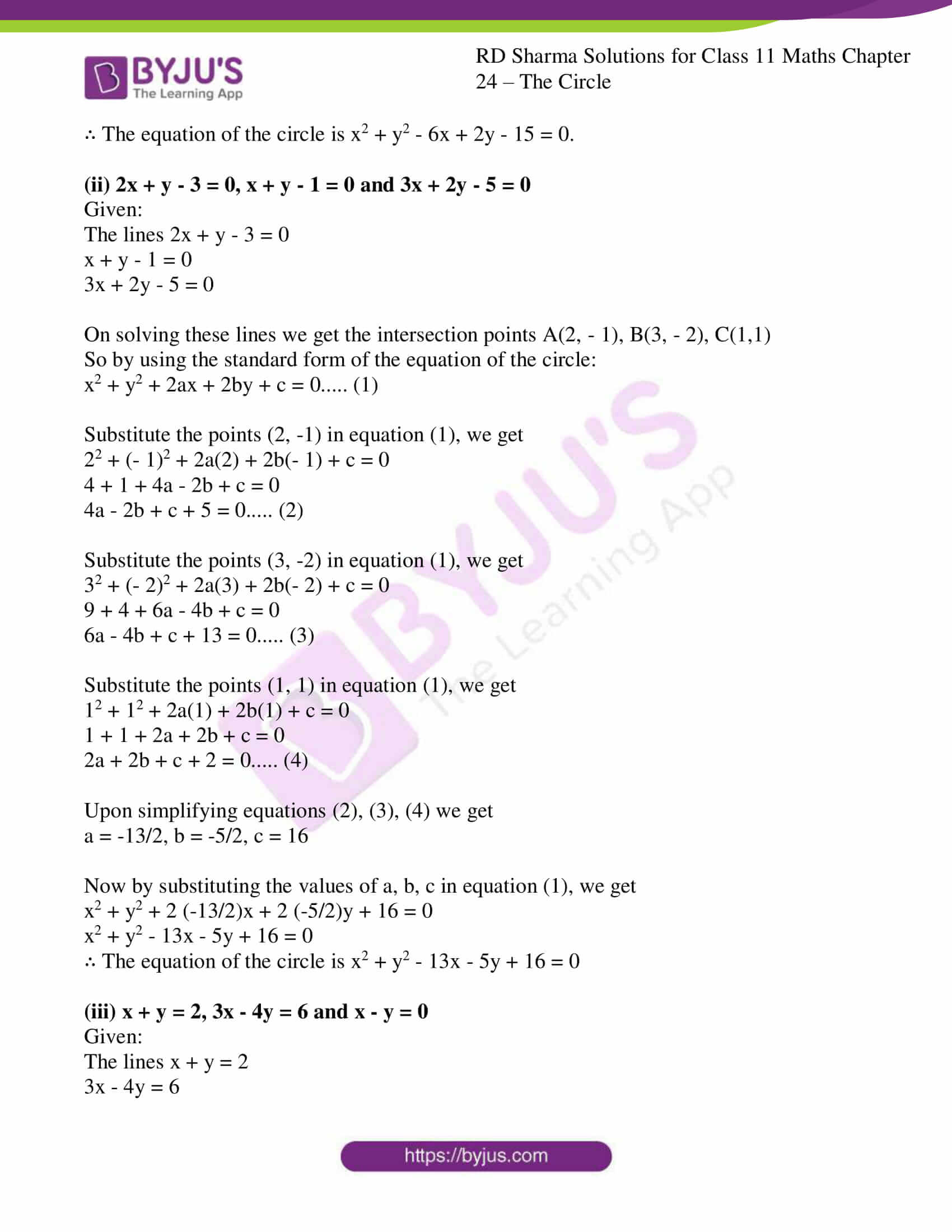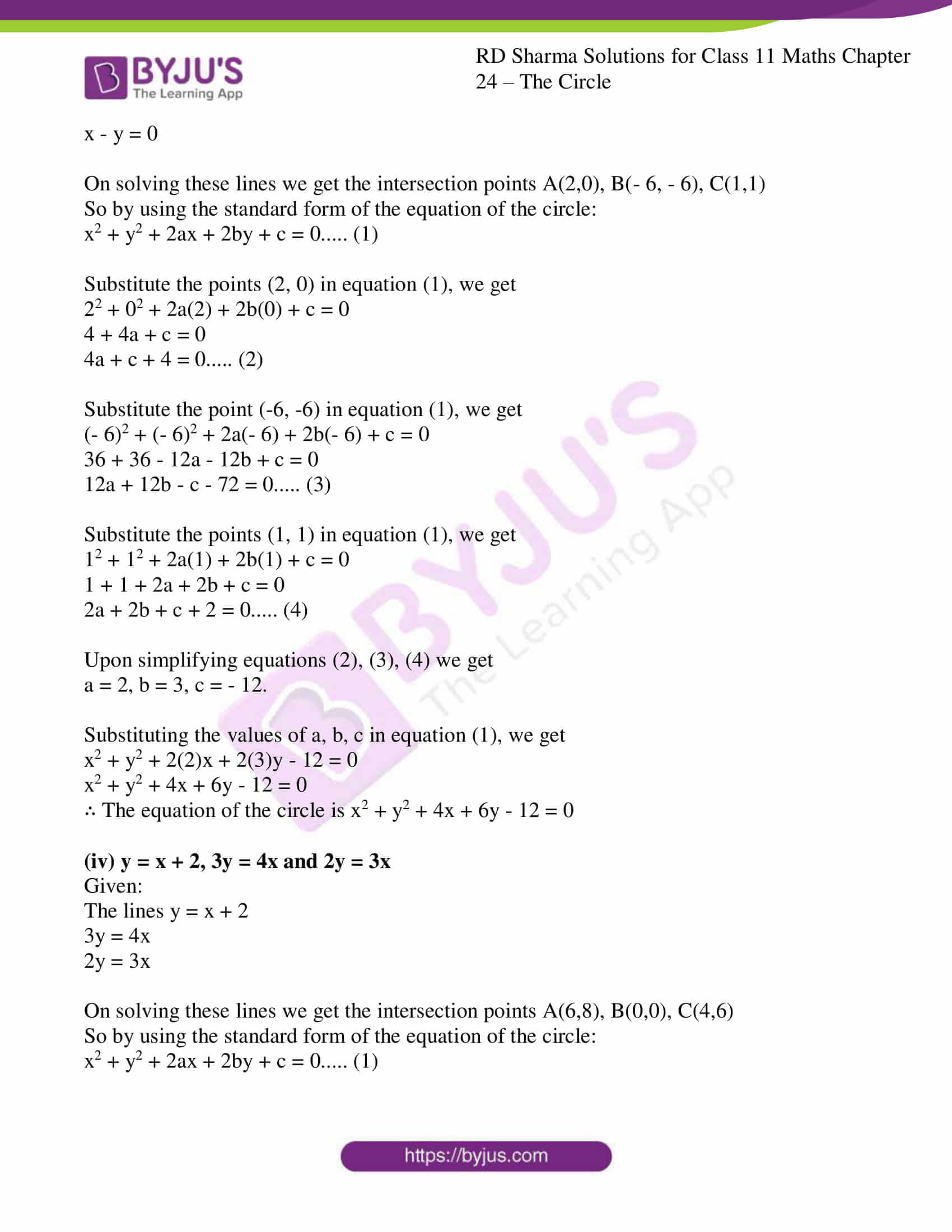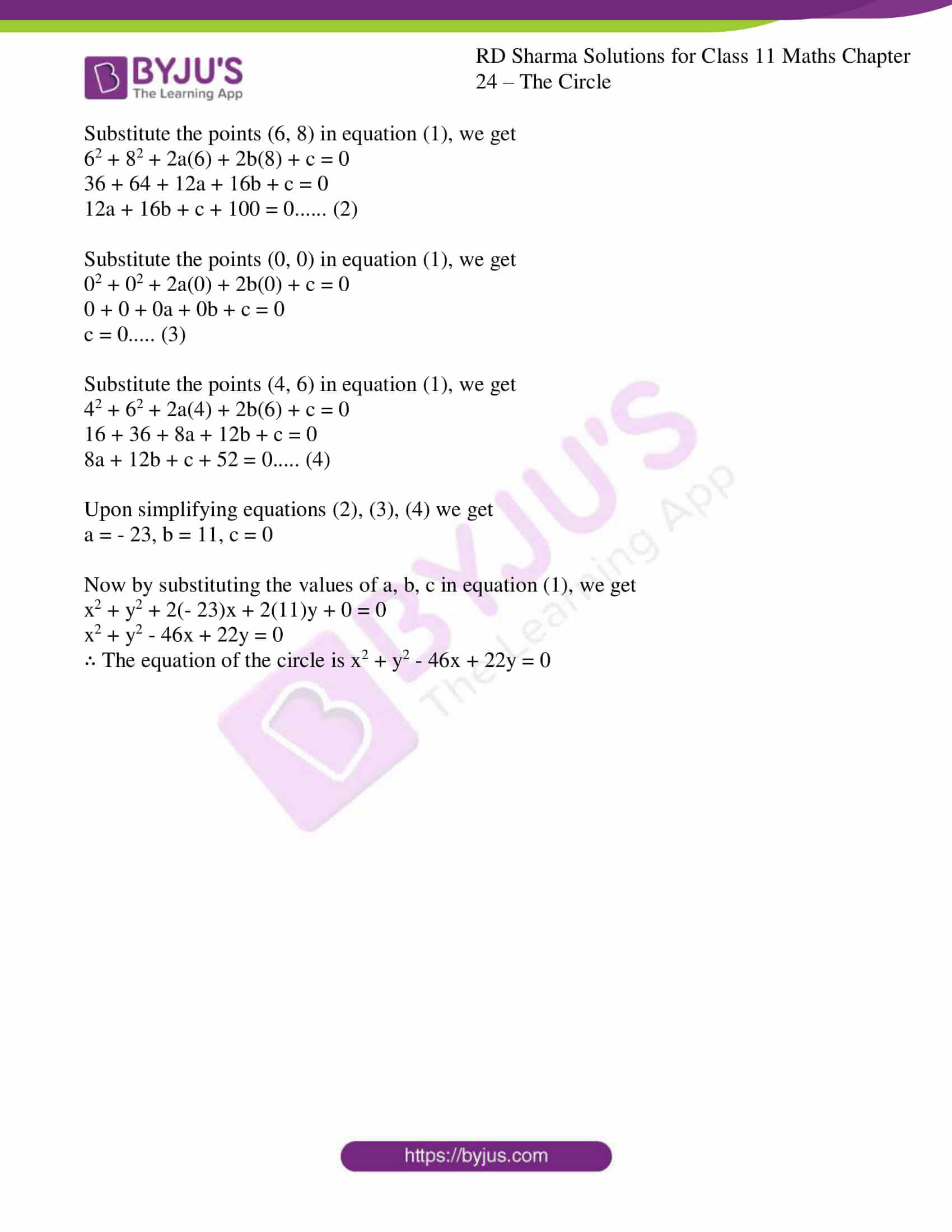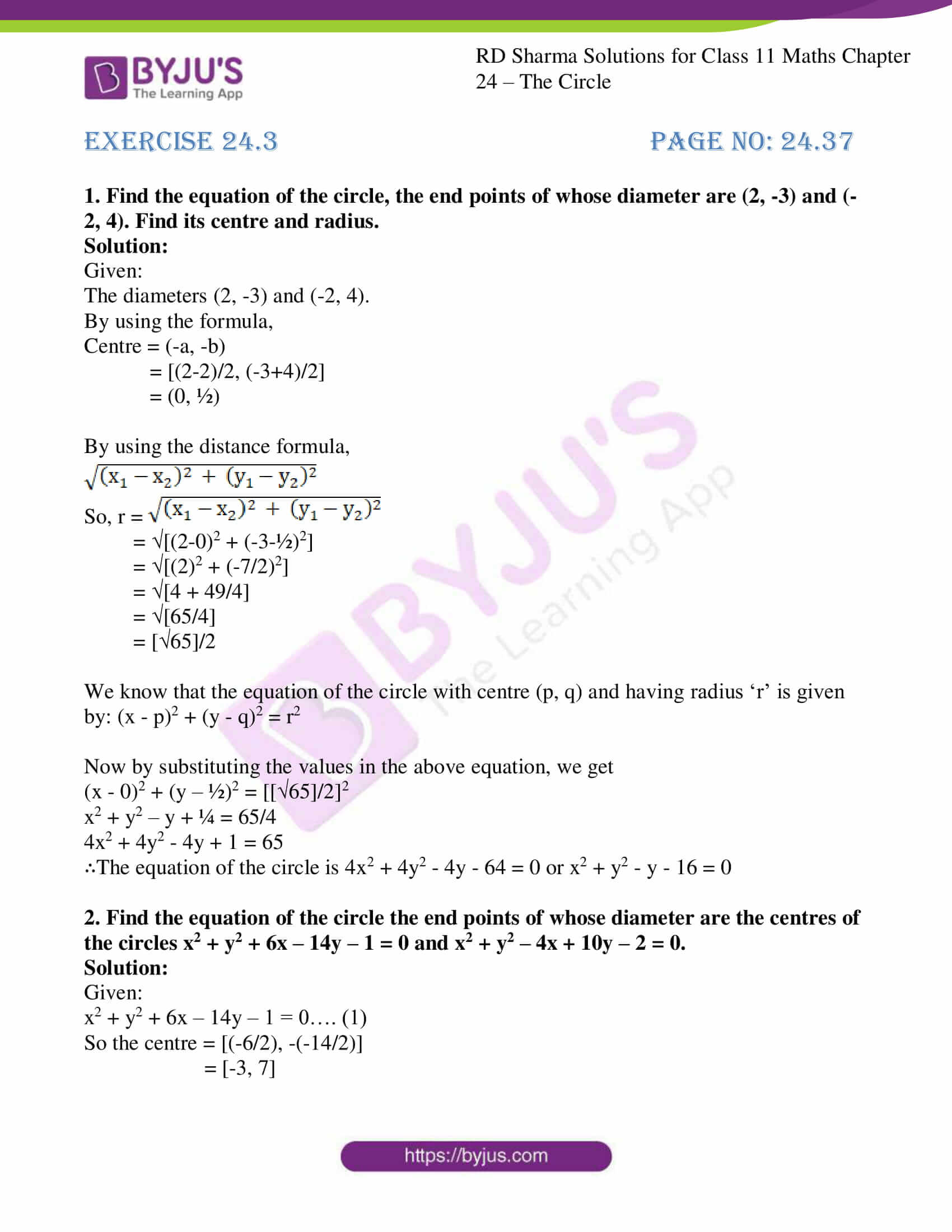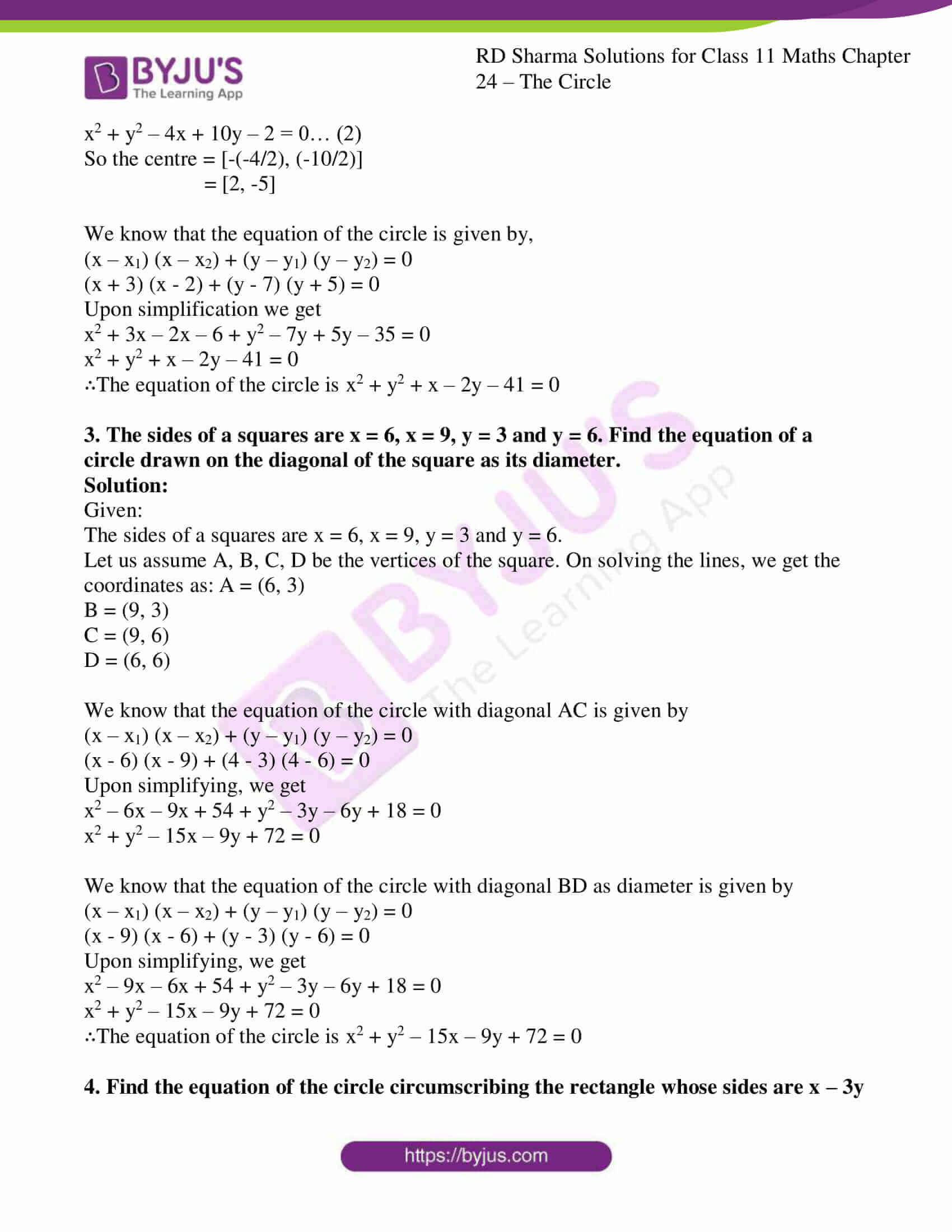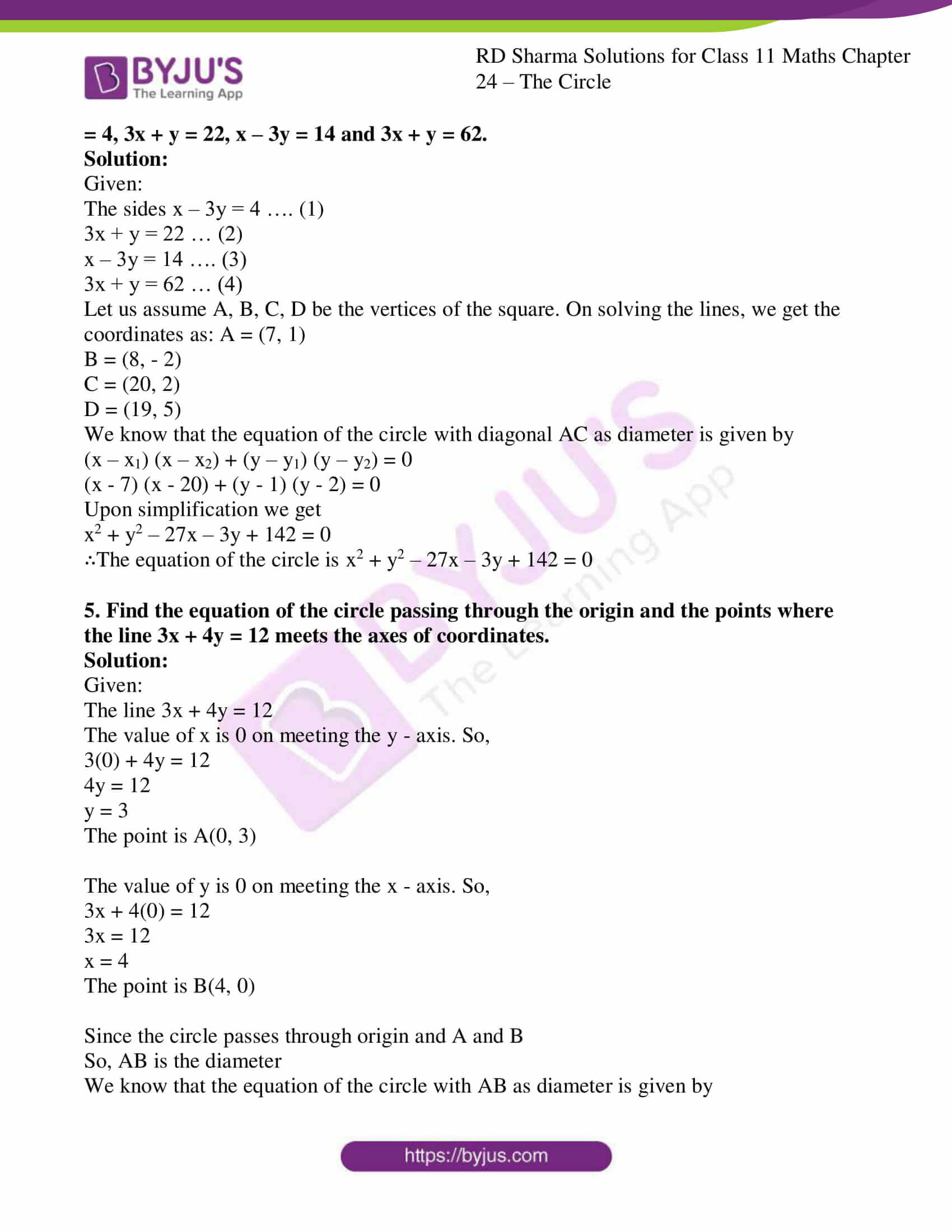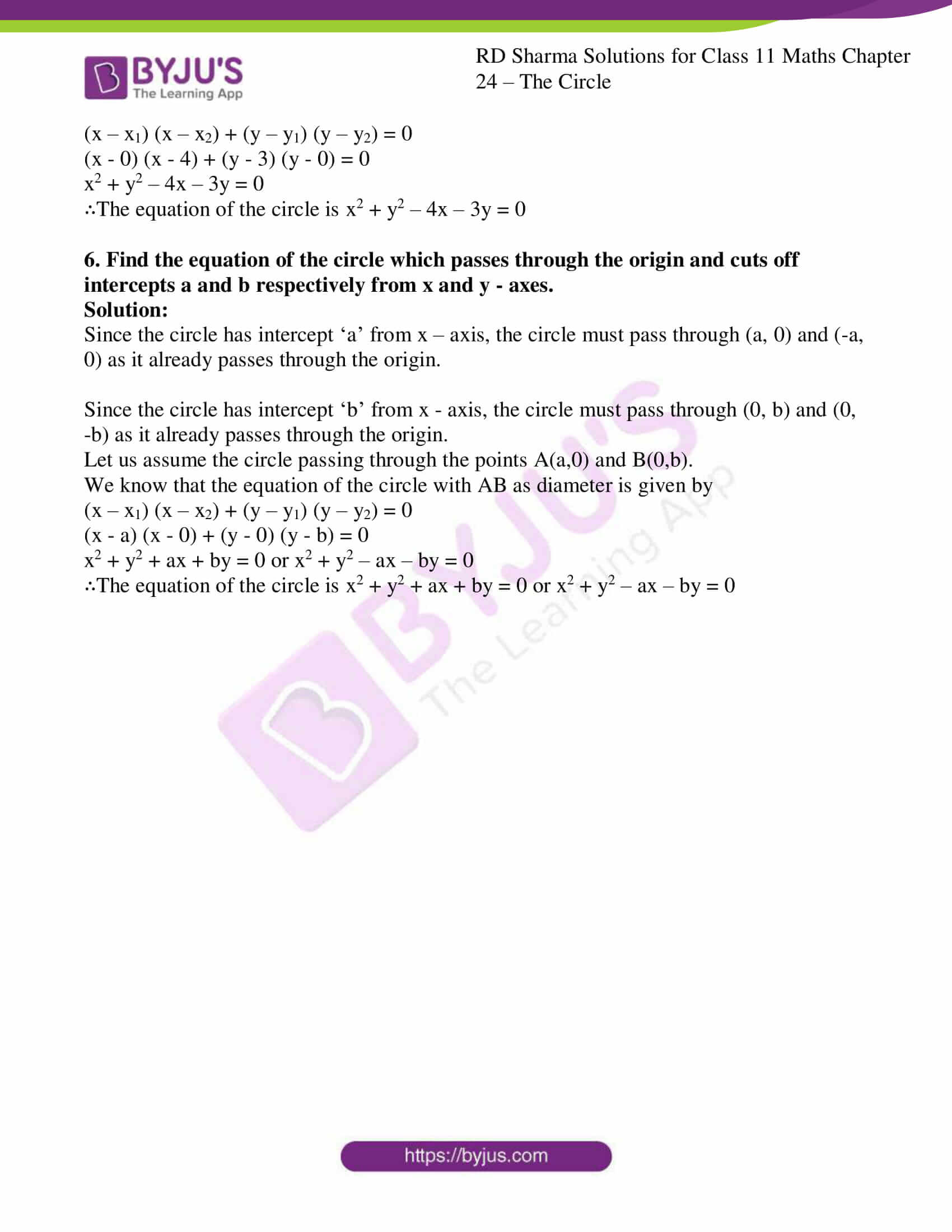### Access answers to RD Sharma Solutions for Class 11 Maths Chapter 24 – The Circle

EXERCISE 24.1 PAGE NO: 24.21

1. Find the equation of the circle with:
(i) Centre (-2, 3) and radius 4.

(ii) Centre (a, b) and radius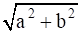.

(iii) Centre (0, – 1) and radius 1.

(iv) Centre (a cos α, a sin α) and radius a.

(v) Centre (a, a) and radius √2 a.

Solution:

(i) Centre (-2, 3) and radius 4.

Given:

The radius is 4 and the centre (-2, 3)

By using the formula,

The equation of the circle with centre (p, q) and radius ‘r’ is (x – p)2 + (y – q)2 = r2

Where, p = -2, q = 3, r = 4

Now by substituting the values in the above equation, we get

(x – p)2 + (y – q)2 = r2

(x – (-2))2 + (y – 3)2 = 42

(x + 2)2 + (y – 3)2 = 16

x2 + 4x + 4 + y2 – 6y + 9 = 16

x2 + y2 + 4x – 6y – 3 = 0

∴ The equation of the circle is x2 + y2 + 4x – 6y – 3 = 0

(ii) Centre (a, b) and radius.

Given:and the centre (a, b)

By using the formula,

The equation of the circle with centre (p, q) and radius ‘r’ is (x – p)2 + (y – q)2 = r2

Where, p = a, q = b, r =Now by substituting the values in the above equation, we get

(x – p)2 + (y – q)2 = r2

(x – a)2 + (y – b)2 =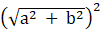x2 – 2ax + a2 + y2 – 2by + b2 = a2 + b2

x2 + y2 – 2ax – 2by = 0

∴ The equation of the circle is x2 + y2 – 2ax – 2by = 0

(iii) Centre (0, -1) and radius 1.

Given:

The radius is 1 and the centre (0, -1)

By using the formula,

The equation of the circle with centre (p, q) and radius ‘r’ is (x – p)2 + (y – q)2 = r2

Where, p = 0, q = -1, r = 1

Now by substituting the values in the above equation, we get

(x – p)2 + (y – q)2 = r2

(x – 0)2 + (y – (-1))2 = 12

(x – 0)2 + (y + 1)2 = 1

x2 + y2 + 2y + 1 = 1

x2 + y2 + 2y = 0

∴ The equation of the circle is x2 + y2 + 2y = 0.

(iv) Centre (a cos α, a sin α) and radius a.

Given:

The radius is ‘a’ and the centre (a cos α, a sin α)

By using the formula,

The equation of the circle with centre (p, q) and radius ‘r’ is (x – p)2 + (y – q)2 = r2

Where, p = a cos α, q = a sin α, r = a

Now by substituting the values in the above equation, we get

(x – p)2 + (y – q)2 = r2

(x – a cosα)2 + (y – a sinα)2 = a2

x2 – (2acosα)x + a2cos2α + y2 – (2asinα)y + a2sin2α = a2

We know that sin2θ + cos2θ = 1

So,

x2 – (2acosα)x + y2 – 2asinαy + a2 = a2

x2 + y2 – (2acosα)x – (2asinα)y = 0

∴ The equation of the circle is x2 + y2 – (2acosα) x – (2asinα) y = 0.

(v) Centre (a, a) and radius √2 a.

Given:

The radius is √2 a and the centre (a, a)

By using the formula,

The equation of the circle with centre (p, q) and radius ‘r’ is (x – p)2 + (y – q)2 = r2

Where, p = a, q = a, r = √2 a

Now by substituting the values in the above equation, we get

(x – p)2 + (y – q)2 = r2

(x – a)2 + (y – a)2 = (√2 a)2

x2 – 2ax + a2 + y2 – 2ay + a2 = 2a2

x2 + y2 – 2ax – 2ay = 0

∴ The equation of the circle is x2 + y2 – 2ax – 2ay = 0.

2. Find the centre and radius of each of the following circles:
(i) (x – 1)2 + y2 = 4

(ii) (x + 5)2 + (y + 1)2 = 9

(iii) x2 + y2 – 4x + 6y = 5

(iv) x2 + y2 – x + 2y – 3 = 0

Solution:

(i) (x – 1)2 + y2 = 4

Given:

The equation (x – 1)2 + y2 = 4

We need to find the centre and the radius.

By using the standard equation formula,

(x – a)2 + (y – b)2 = r2 …. (1)

Now let us convert given circle’s equation into the standard form.

(x – 1)2 + y2 = 4

(x – 1)2 + (y – 0)2 = 22 ….. (2)

By comparing equation (2) with (1), we get

Centre = (1, 0) and radius = 2

∴ The centre of the circle is (1, 0) and the radius is 2.

(ii) (x + 5)2 + (y + 1)2 = 9

Given:

The equation (x + 5)2 + (y + 1)2 = 9

We need to find the centre and the radius.

By using the standard equation formula,

(x – a)2 + (y – b)2 = r2 …. (1)

Now let us convert given circle’s equation into the standard form.

(x + 5)2 + (y + 1)2 = 9

(x – (-5))2 + (y – ( – 1))2 = 32 …. (2)

By comparing equation (2) with (1), we get

Centre = (-5, -1) and radius = 3

∴ The centre of the circle is (-5, -1) and the radius is 3.

(iii) x2 + y2 – 4x + 6y = 5

Given:

The equation x2 + y2 – 4x + 6y = 5

We need to find the centre and the radius.

By using the standard equation formula,

(x – a)2 + (y – b)2 = r2 …. (1)

Now let us convert given circle’s equation into the standard form.

x2 + y2 – 4x + 6y = 5

(x2 – 4x + 4) + (y2 + 6y + 9) = 5 + 4 + 9

(x – 2)2 + (y + 3)2 = 18

(x – 2)2 + (y – (-3))2 = (3√2)2 … (2)

By comparing equation (2) with (1), we get

Centre = (2, -3) and radius = 3√2

∴ The centre of the circle is (2, -3) and the radius is 3√2.

(iv) x2 + y2 – x + 2y – 3 = 0

Given:

The equation x2 + y2 – x + 2y – 3 = 0

We need to find the centre and the radius.

By using the standard equation formula,

(x – a)2 + (y – b)2 = r2 …. (1)

Now let us convert given circle’s equation into the standard form.

x2 + y2 – x + 2y – 3 = 0

(x2 – x + ¼) + (y2 + 2y + 1) – 3 – ¼ – 1 = 0

(x – ½)2 + (y + 1)2 = 17/4 …. (2)

By comparing equation (2) with (1), we get

Centre = (½, – 1) and radius = √17/2

∴ The centre of the circle is (½, -1) and the radius is √17/2.

3. Find the equation of the circle whose centre is (1, 2) and which passes through the point (4, 6).

Solution:

Given:

Centre is (1, 2) and which passes through the point (4, 6).

Where, p = 1, q = 2

We need to find the equation of the circle.

By using the formula,

(x – p)2 + (y – q)2 = r2

(x – 1)2 + (y – 2)2 = r2

It passes through the point (4, 6)

(4 – 1)2 + (6 – 2)2 = r2

32 + 42 = r2

9 + 16 = r2

25 = r2

r = √25

= 5

So r = 5 units

We know that the equation of the circle with centre (p, q) and having radius ‘r’ is given by: (x – p)2 + (y – q)2 = r2

By substitute the values in the above equation, we get

(x – 1)2 + (y – 2)2 = 52

x2 – 2x + 1 + y2 – 4y + 4 = 25

x2 + y2 – 2x – 4y – 20 = 0.

∴ The equation of the circle is x2 + y2 – 2x – 4y – 20 = 0.

4. Find the equation of the circle passing through the point of intersection of the lines x + 3y = 0 and 2x – 7y = 0 and whose centre is the point of intersection of the lines x + y + 1 = 0 and x – 2y + 4 = 0.

Solution:

Let us find the points of intersection of the lines.

On solving the lines x + 3y = 0 and 2x – 7y = 0, we get the point of intersection to be (0, 0)

On solving the lines x + y + 1 and x – 2y + 4 = 0, we get the point of intersection to be (-2, 1)

We have circle with centre (-2, 1) and passing through the point (0, 0).

We know that the radius of the circle is the distance between the centre and any point on the radius. So, we find the radius of the circle.

So, the equation is (x – p)2 + (y – q)2 = r2

Where, p = -2, q = 1

(x + 2)2 + (y – 1)2 = r2 …. (1)

Equation (1) passes through (0, 0)

So, (0 + 2)2 + (0 – 1)2 = r2

4 + 1 = a2

5 = r2

r = √5

We know that the equation of the circle with centre (p, q) and having radius ‘r’ is given by: (x – p)2 + (y – q)2 = r2

By substitute the values in the above equation, we get

(x – (-2))2 + (y – 1)2 = (√5)2

(x + 2)2 + (y – 1)2 = 5

x2 + 4x + 4 + y2 – 2y + 1 = 5

x2 + y2 + 4x – 2y = 0

∴ The equation of the circle is x2 + y2 + 4x – 2y = 0.

5. Find the equation of the circle whose centre lies on the positive direction of y – axis at a distance 6 from the origin and whose radius is 4.

Solution:

It is given that the centre lies on the positive y – axis at a distance of 6 from the origin, we get the centre (0, 6).

We have a circle with centre (0, 6) and having radius 4.

We know that the equation of the circle with centre (p, q) and having radius ‘r’ is given by: (x – p)2 + (y – q)2 = r2

Where, p = 0, q = 6, r = 4

Now by substituting the values in the equation, we get

(x – 0)2 + (y – 6)2 = 42

x2 + y2 – 12y + 36 = 16

x2 + y2 – 12y + 20 = 0.

∴ The equation of the circle is x2 + y2 – 12y + 20 = 0.

6. If the equations of two diameters of a circle are 2x + y = 6 and 3x + 2y = 4 and the radius is 10, find the equation of the circle.

Solution:

It is given that the circle has the radius 10 and has diameters 2x + y = 6 and 3x + 2y = 4.

We know that the centre is the intersection point of the diameters.

On solving the diameters, we get the centre to be (8, -10).

We have a circle with centre (8, -10) and having radius 10.

By using the formula,

We know that the equation of the circle with centre (p, q) and having radius ‘r’ is given by: (x – p)2 + (y – q)2 = r2

Where, p = 8, q = -10, r = 10

Now by substituting the values in the equation, we get

(x – 8)2 + (y – (-10))2 = 102

(x – 8)2 + (y + 10)2 = 100

x2 – 16x + 64 + y2 + 20y + 100 = 100

x2 + y2 – 16x + 20y + 64 = 0.

∴ The equation of the circle is x2 + y2 – 16x + 20y + 64 = 0.

7. Find the equation of the circle
(i) which touches both the axes at a distance of 6 units from the origin.

(ii) Which touches x – axis at a distance of 5 from the origin and radius 6 units.

(iii) Which touches both the axes and passes through the point (2, 1).

(iv) Passing through the origin, radius 17 and ordinate of the centre is – 15.

Solution:

(i) which touches both the axes at a distance of 6 units from the origin.

A circle touches the axes at the points (±6, 0) and (0, ±6).

So, a circle has a centre (±6, ±6) and passes through the point (0, 6).

We know that the radius of the circle is the distance between the centre and any point on the radius. So, we find the radius of the circle.

So, the equation is (x – p)2 + (y – q)2 = r2

Where, p = 6, q = 6

(x – 6)2 + (y – 6)2 = r2 …. (1)

Equation (1) passes through (0, 6)

So, (0 – 6)2 + (6 – 6)2 = r2

36 + 0 = r2

r = √36

= 6

We know that the equation of the circle with centre (p, q) and having radius ‘r’ is given by: (x – p)2 + (y – q)2 = r2

Now by substituting the values in the equation, we get

(x ± 6)2 + (y ± 6)2 = (6)2

x2 ± 12x + 36 + y2 ± 12y + 36 = 36

x2 + y2 ± 12x ± 12y + 36 = 0

∴ The equation of the circle is x2 + y2 ± 12x ± 12y + 36 = 0.

(ii) Which touches x – axis at a distance of 5 from the origin and radius 6 units.

A circle touches the x – axis at the points (±5, 0).

Let us assume the centre of the circle is (±5, a).

We have a circle with centre (5, a) and passing through the point (5, 0) and having radius 6.

We know that the radius of the circle is the distance between the centre and any point on the radius. So, we find the radius of the circle.

So, the equation is (x – p)2 + (y – q)2 = r2

Where, p = 5, q = a

(x – 5)2 + (y – a)2 = r2 …. (1)

Equation (1) passes through (5, 0)

So, (5 – 5)2 + (0 – 6)2 = r2

0 + 36 = r2

r = √36

= 6

We have got the centre at (±5, ±6) and having radius 6 units.

We know that the equation of the circle with centre (p, q) and having radius ‘r’ is given by: (x – p)2 + (y – q)2 = r2

Now by substituting the values in the equation, we get

(x ± 5)2 + (y ± 6)2 = (6)2

x2 ± 10x + 25 + y2 ± 12y + 36 = 36

x2 + y2 ± 10x ± 12y + 25 = 0.

∴ The equation of the circle is x2 + y2 ± 10x ± 12y + 25 = 0.

(iii) Which touches both the axes and passes through the point (2, 1).

Let us assume the circle touches the x-axis at the point (a, 0) and y-axis at the point (0, a).

Then the centre of the circle is (a, a) and radius is a.

Its equation will be (x – p)2 + (y – q)2 = r2

By substituting the values we get

(x – a)2 + (y – a)2 = a2 … (1)

So now, equation (1) passes through P (2, 1)

By substituting the values we get

(2 – a)2 + (1 – a)2 = a2

4 – 4a + a2 + 1 – 2a + a2 = a2

5 – 6a + a2 = 0

(a – 5) (a – 1) = 0

So, a = 5 or 1

Case (i)

We have got the centre at (5, 5) and having radius 5 units.

We know that the equation of the circle with centre (p, q) and having radius ‘r’ is given by: (x – p)2 + (y – q)2 = r2

Now by substituting the values in the equation we get

(x – 5)2 + (y – 5)2 = 52

x2 – 10x + 25 + y2 – 10y + 25 = 25

x2 + y2 – 10x – 10y + 25 = 0.

∴ The equation of the circle is x2 + y2 – 10x – 10y + 25 = 0.

Case (ii)

We have got the centre at (1, 1) and having a radius 1 unit.

We know that the equation of the circle with centre (p, q) and having radius ‘r’ is given by: (x – p)2 + (y – q)2 = r2

Now by substituting the values in the equation we get

(x – 1)2 + (y – 1)2 = 12

x2 – 2x + 1 + y2 – 2y + 1 = 1

x2 + y2 – 2x – 2y + 1 = 0

∴ The equation of the circle is x2 + y2 – 2x – 2y + 1 = 0.

(iv) Passing through the origin, radius 17 and ordinate of the centre is – 15.

Let us assume the abscissa as ‘a’

We have a circle with centre (a, – 15) and passing through the point (0, 0) and having radius 17.

We know that the radius of the circle is the distance between the centre and any point on the radius. So, we find the radius of the circle.

By using the distance formula,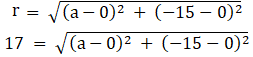172 = a2 + (-15)2

289 = a2 + 225

a2 = 64

|a| = √64

|a| = 8

a = ±8 …. (1)

We have got the centre at (±8, – 15) and having radius 17 units.

We know that the equation of the circle with centre (p, q) and having radius ‘r’ is given by: (x – p)2 + (y – q)2 = r2

Now by substituting the values in the equation, we get

(x ± 8)2 + (y – 15)2 = 172

x2 ± 16x + 64 + y2 – 30y + 225 = 289

x2 + y2 ± 16x – 30y = 0.

∴ The equation of the circle is x2 + y2 ± 16x – 30y = 0.

8. Find the equation of the circle which has its centre at the point (3, 4) and touches the straight line 5x + 12y – 1 = 0.

Solution:

It is given that we need to find the equation of the circle with centre (3, 4) and touches the straight line 5x + 12y – 1 = 0.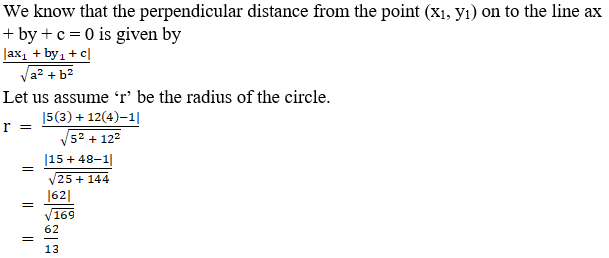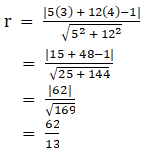We have a circle with centre (3, 4) and having a radius 62/13.

We know that the equation of the circle with centre (p, q) and having radius ‘r’ is given by: (x – p)2 + (y – q)2 = r2

Now by substituting the values in the equation, we get

(x – 3)2 + (y – 4)2 = (62/13)2

x2 – 6x + 9 + y2 – 8y + 16 = 3844/169

169x2 + 169y2 – 1014x – 1352y + 4225 = 3844

169x2 + 169y2 – 1014x – 1352y + 381 = 0

∴ The equation of the circle is 169x2 + 169y2 – 1014x – 1352y + 381 = 0.

9. Find the equation of the circle which touches the axes and whose centre lies on x – 2y = 3.

Solution:

Let us assume the circle touches the axes at (a, 0) and (0, a) and we get the radius to be |a|.

We get the centre of the circle as (a, a). This point lies on the line x – 2y = 3

a – 2(a) = 3

-a = 3

a = – 3

Centre = (a, a) = (-3, -3) and radius of the circle(r) = |-3| = 3

We have circle with centre (-3, -3) and having radius 3.

We know that the equation of the circle with centre (p, q) and having radius ‘r’ is given by: (x – p)2 + (y – q)2 = r2

Now by substituting the values in the equation, we get

(x – (-3))2 + (y – (-3))2 = 32

(x + 3)2 + (y + 3)2 = 9

x2 + 6x + 9 + y2 + 6y + 9 = 9

x2 + y2 + 6x + 6y + 9 = 0

∴ The equation of the circle is x2 + y2 + 6x + 6y + 9 = 0.

10. A circle whose centre is the point of intersection of the lines 2x – 3y + 4 = 0 and 3x + 4y – 5 = 0 passes through the origin. Find its equation.

Solution:

It is given that the circle has the centre at the intersection point of the lines 2x – 3y + 4 = 0 and 3x + 4y – 5 = 0 and passes through the origin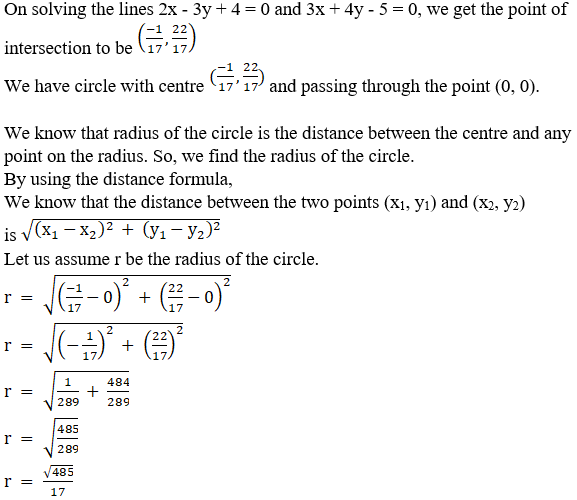We know that the equation of the circle with centre (p, q) and having radius ‘r’ is given by: (x – p)2 + (y – q)2 = r2

Now by substituting the values in the equation, we get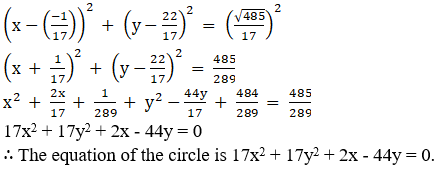EXERCISE 24.2 PAGE NO: 24.31

1. Find the coordinates of the centre radius of each of the following circle:
(i) x2 + y2 + 6x – 8y – 24 = 0

(ii) 2x2 + 2y2 – 3x + 5y = 7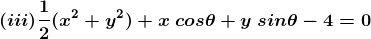(iv) x2 + y2 – ax – by = 0

Solution:

(i) x2 + y2 + 6x – 8y – 24 = 0

Given:

The equation of the circle is x2 + y2 + 6x – 8y – 24 = 0 …… (1)

We know that for a circle x2 + y2 + 2ax + 2by + c = 0 …… (2)

Centre = (-a, -b)

So by comparing equation (1) and (2)

Centre = (-6/2, -(-8)/2)

= (-3, 4)

Radius = (a2 + b2 – c)

= (32 + 42 – (-24))

= (9 + 16 + 24)

= (49)

= 7

∴ The centre of the circle is (-3, 4) and the radius is 7.

(ii) 2x2 + 2y2 – 3x + 5y = 7

Given:

The equation of the circle is 2x2 + 2y2 – 3x + 5y = 7 (divide by 2 we get)

x2 + y2 – 3x/2 + 5y/2 = 7/2

Now, by comparing with the equation x2 + y2 + 2ax + 2by + c = 0

Centre = (-a, -b)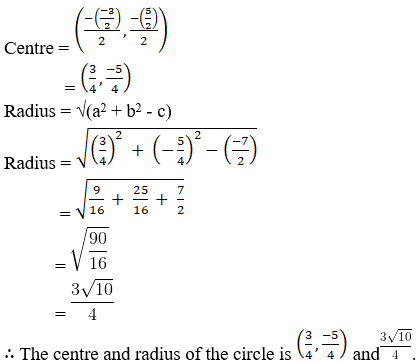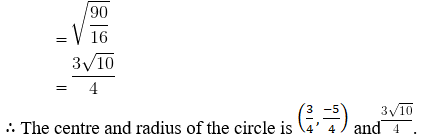Given:

The equation of the circle is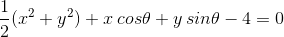(Multiply by 2 we get)

x2 + y2 + 2x cos θ + 2y sin θ – 8 = 0

By comparing with the equation x2 + y2 + 2ax + 2by + c = 0

Centre = (-a, -b)

= [(-2cos θ)/2 , (-2sin θ)/2]

= (-cos θ, -sin θ)

Radius = (a2 + b2 – c)

= √[(-cos θ)2 + (sin θ)2 – (-8)]

= √[cos2 θ + sin2 θ + 8]

= √[1 + 8]

= √

= 3

∴ The centre and radius of the circle is (-cos θ, -sin θ) and 3.

(iv) x2 + y2 – ax – by = 0

Given:

Equation of the circle is x2 + y2 – ax – by = 0

By comparing with the equation x2 + y2 + 2ax + 2by + c = 0

Centre = (-a, -b)

= (-(-a)/2, -(-b)/2)

= (a/2, b/2)

Radius = (a2 + b2 – c)

= √[(a/2)2 + (b/2)2]

= [(a2/4 + b2/4)]

= [(a2 + b2)/4]

= [(a2 + b2)]/2

∴ The centre and radius of the circle is (a/2, b/2) and [(a2 + b2)]/2

2. Find the equation of the circle passing through the points :
(i) (5, 7), (8, 1) and (1, 3)

(ii) (1, 2), (3, – 4) and (5, – 6)

(iii) (5, -8), (-2, 9) and (2, 1)

(iv) (0, 0), (-2, 1) and (-3, 2)

Solution:

(i) (5, 7), (8, 1) and (1, 3)

By using the standard form of the equation of the circle:

x2 + y2 + 2ax + 2by + c = 0 ….. (1)

Firstly let us find the values of a, b and c

Substitute the given point (5, 7) in equation (1), we get

52 + 72 + 2a (5) + 2b (7) + c = 0

25 + 49 + 10a + 14b + c = 0

10a + 14b + c + 74 = 0….. (2)

By substituting the given point (8, 1) in equation (1), we get

82 + 12 + 2a (8) + 2b (1) + c = 0

64 + 1 + 16a + 2b + c = 0

16a + 2b + c + 65 = 0….. (3)

Substituting the point (1, 3) in equation (1), we get

12 + 32 + 2a (1) + 2b (3) + c = 0

1 + 9 + 2a + 6b + c = 0

2a + 6b + c + 10 = 0….. (4)

Now by simplifying the equations (2), (3), (4) we get the values

a = -29/6, b = -19/6, c = 56/3

Substituting the values of a, b, c in equation (1), we get

x2 + y2 + 2 (-29/6)x + 2 (-19/6) + 56/3 = 0

x2 + y2 – 29x/3 – 19y/3 + 56/3 = 0

3x2 + 3y2 – 29x – 19y + 56 = 0

∴ The equation of the circle is 3x2 + 3y2 – 29x – 19y + 56 = 0

(ii) (1, 2), (3, – 4) and (5, – 6)

By using the standard form of the equation of the circle:

x2 + y2 + 2ax + 2by + c = 0 ….. (1)

Substitute the points (1, 2) in equation (1), we get

12 + 22 + 2a (1) + 2b (2) + c = 0

1 + 4 + 2a + 4b + c = 0

2a + 4b + c + 5 = 0….. (2)

Substitute the points (3, -4) in equation (1), we get

32 + (- 4)2 + 2a (3) + 2b (- 4) + c = 0

9 + 16 + 6a – 8b + c = 0

6a – 8b + c + 25 = 0….. (3)

Substitute the points (5, -6) in equation (1), we get

52 + (- 6)2 + 2a (5) + 2b (- 6) + c = 0

25 + 36 + 10a – 12b + c = 0

10a – 12b + c + 61 = 0….. (4)

Now by simplifying the equations (2), (3), (4) we get

a = – 11, b = – 2, c = 25

Substitute the values of a, b and c in equation (1), we get

x2 + y2 + 2(- 11)x + 2(- 2) + 25 = 0

x2 + y2 – 22x – 4y + 25 = 0

∴ The equation of the circle is x2 + y2 – 22x – 4y + 25 = 0

(iii) (5, -8), (-2, 9) and (2, 1)

By using the standard form of the equation of the circle:

x2 + y2 + 2ax + 2by + c = 0 ….. (1)

Substitute the point (5, -8) in equation (1), we get

52 + (- 8)2 + 2a(5) + 2b(- 8) + c = 0

25 + 64 + 10a – 16b + c = 0

10a – 16b + c + 89 = 0….. (2)

Substitute the points (-2, 9) in equation (1), we get

(- 2)2 + 92 + 2a(- 2) + 2b(9) + c = 0

4 + 81 – 4a + 18b + c = 0

-4a + 18b + c + 85 = 0….. (3)

Substitute the points (2, 1) in equation (1), we get

22 + 12 + 2a(2) + 2b(1) + c = 0

4 + 1 + 4a + 2b + c = 0

4a + 2b + c + 5 = 0….. (4)

By simplifying equations (2), (3), (4) we get

a = 58, b = 24, c = – 285.

Now, by substituting the values of a, b, c in equation (1), we get

x2 + y2 + 2(58)x + 2(24) – 285 = 0

x2 + y2 + 116x + 48y – 285 = 0

∴ The equation of the circle is x2 + y2 + 116x + 48y – 285 = 0

(iv) (0, 0), (-2, 1) and (-3, 2)

By using the standard form of the equation of the circle:

x2 + y2 + 2ax + 2by + c = 0 ….. (1)

Substitute the points (0, 0) in equation (1), we get

02 + 02 + 2a(0) + 2b(0) + c = 0

0 + 0 + 0a + 0b + c = 0

c = 0….. (2)

Substitute the points (-2, 1) in equation (1), we get

(- 2)2 + 12 + 2a(- 2) + 2b(1) + c = 0

4 + 1 – 4a + 2b + c = 0

-4a + 2b + c + 5 = 0….. (3)

Substitute the points (-3, 2) in equation (1), we get

(- 3)2 + 22 + 2a(- 3) + 2b(2) + c = 0

9 + 4 – 6a + 4b + c = 0

-6a + 4b + c + 13 = 0….. (4)

By simplifying the equations (2), (3), (4) we get

a = -3/2, b = -11/2, c = 0

Now, by substituting the values of a, b, c in equation (1), we get

x2 + y2 + 2(-3/2)x + 2(-11/2)y + 0 = 0

x2 + y2 – 3x – 11y = 0

∴ The equation of the circle is x2 + y2 – 3x – 11y = 0

3. Find the equation of the circle which passes through (3, – 2), (- 2, 0) and has its centre on the line 2x – y = 3.

Solution:

Given:

The line 2x – y = 3 … (1)

The points (3, -2), (-2, 0)

By using the standard form of the equation of the circle:

x2 + y2 + 2ax + 2by + c = 0 ….. (2)

Let us substitute the centre (-a, -b) in equation (1) we get,

2(- a) – (- b) = 3

-2a + b = 3

2a – b + 3 = 0…… (3)

Now Substitute the given points (3, -2) in equation (2), we get

32 + (- 2)2 + 2a(3) + 2b(- 2) + c = 0

9 + 4 + 6a – 4b + c = 0

6a – 4b + c + 13 = 0….. (4)

Substitute the points (-2, 0) in equation (2), we get

(- 2)2 + 02 + 2a(- 2) + 2b(0) + c = 0

4 + 0 – 4a + c = 0

4a – c – 4 = 0….. (5)

By simplifying the equations (3), (4) and (5) we get,

a = 3/2, b = 6, c = 2

Again by substituting the values of a, b, c in (2), we get

x2 + y2 + 2 (3/2)x + 2 (6)y + 2 = 0

x2 + y2 + 3x + 12y + 2 = 0

∴ The equation of the circle is x2 + y2 + 3x + 12y + 2 = 0.

4. Find the equation of the circle which passes through the points (3, 7), (5, 5) and has its centre on line x – 4y = 1.

Solution:

Given:

The points (3, 7), (5, 5)

The line x – 4y = 1…. (1)

By using the standard form of the equation of the circle:

x2 + y2 + 2ax + 2by + c = 0 ….. (2)

Let us substitute the centre (-a, -b) in equation (1) we get,

(- a) – 4(- b) = 1

-a + 4b = 1

a – 4b + 1 = 0…… (3)

Substitute the points (3, 7) in equation (2), we get

32 + 72 + 2a(3) + 2b(7) + c = 0

9 + 49 + 6a + 14b + c = 0

6a + 14b + c + 58 = 0….. (4)

Substitute the points (5, 5) in equation (2), we get

52 + 52 + 2a(5) + 2b(5) + c = 0

25 + 25 + 10a + 10b + c = 0

10a + 10b + c + 50 = 0….. (5)

By simplifying equations (3), (4) and (5) we get,

a = 3, b = 1, c = – 90

Now, by substituting the values of a, b, c in equation (2), we get

x2 + y2 + 2(3)x + 2(1)y – 90 = 0

x2 + y2 + 6x + 2y – 90 = 0

∴ The equation of the circle is x2 + y2 + 6x + 2y – 90 = 0.

5. Show that the points (3, -2), (1, 0), (-1, -2) and (1, -4) are con – cyclic.

Solution:

Given:

The points (3, -2), (1, 0), (-1, -2) and (1, -4)

Let us assume the circle passes through the points A, B, C.

So by using the standard form of the equation of the circle:

x2 + y2 + 2ax + 2by + c = 0….. (1)

Substitute the points A (3, – 2) in equation (1), we get,

32 + (-2)2 + 2a(3) + 2b(-2) + c = 0

9 + 4 + 6a – 4b + c = 0

6a – 4b + c + 13 = 0….. (2)

Substitute the points B (1, 0) in equation (1), we get,

12 + 02 + 2a(1) + 2b(0) + c = 0

1 + 2a + c = 0 ……- (3)

Substitute the points C (-1, -2) in equation (1), we get,

(- 1)2 + (- 2)2 + 2a(- 1) + 2b(- 2) + c = 0

1 + 4 – 2a – 4b + c = 0

5 – 2a – 4b + c = 0

2a + 4b – c – 5 = 0….. (4)

Upon simplifying the equations (2), (3) and (4) we get,

a = – 1, b = 2 and c = 1

Substituting the values of a, b, c in equation (1), we get

x2 + y2 + 2(- 1)x + 2(2)y + 1 = 0

x2 + y2 – 2x + 4y + 1 = 0 ….. (5)

Now by substituting the point D (1, -4) in equation (5) we get,

12 + (- 4)2 – 2(1) + 4(- 4) + 1

1 + 16 – 2 – 16 + 1

0

∴ The points (3, -2), (1, 0), (-1, -2), (1, -4) are con – cyclic.

6. Show that the points (5, 5), (6, 4), (- 2, 4) and (7, 1) all lie on a circle, and find its equation, centre, and radius.

Solution:

Given:

The points (5, 5), (6, 4), (- 2, 4) and (7, 1) all lie on a circle.

Let us assume the circle passes through the points A, B, C.

So by using the standard form of the equation of the circle:

x2 + y2 + 2ax + 2by + c = 0….. (1)

Substituting A (5, 5) in (1), we get,

52 + 52 + 2a(5) + 2b(5) + c = 0

25 + 25 + 10a + 10b + c = 0

10a + 10b + c + 50 = 0….. (2)

Substitute the points B (6, 4) in equation (1), we get,

62 + 42 + 2a(6) + 2b(4) + c = 0

36 + 16 + 12a + 8b + c = 0

12a + 8b + c + 52 = 0….. (3)

Substitute the point C (-2, 4) in equation (1), we get,

(-2)2 + 42 + 2a(-2) + 2b(4) + c = 0

4 + 16 – 4a + 8b + c = 0

20 – 4a + 8b + c = 0

4a – 8b – c – 20 = 0….. (4)

Upon simplifying equations (2), (3) and (4) we get,

a = – 2, b = – 1 and c = – 20

Now by substituting the values of a, b, c in equation (1), we get

x2 + y2 + 2(- 2)x + 2(- 1)y – 20 = 0

x2 + y2 – 4x – 2y – 20 = 0 ….. (5)

Substituting D (7, 1) in equation (5) we get,

72 + 12 – 4(7) – 2(1) – 20

49 + 1 – 28 – 2 – 20

0

∴ The points (3, -2), (1, 0), (-1, -2), (1, -4) lie on a circle.

Now let us find the centre and the radius.

We know that for a circle x2 + y2 + 2ax + 2by + c = 0,

Centre = (-a, -b)

Radius = (a2 + b2 – c)

Comparing equation (5) with equation (1), we get

Centre = [-(-4)/2, -(-2)/2)]

= (2, 1)

Radius = (22 + 12 – (-20))

= (25)

= 5

∴ The centre and radius of the circle is (2, 1) and 5.

7. Find the equation of the circle which circumscribes the triangle formed by the lines:
(i) x + y + 3 = 0, x – y + 1 = 0 and x = 3

(ii) 2x + y – 3 = 0, x + y – 1 = 0 and 3x + 2y – 5 = 0

(iii) x + y = 2, 3x – 4y = 6 and x – y = 0

(iv) y = x + 2, 3y = 4x and 2y = 3x

Solution:

(i) x + y + 3 = 0, x – y + 1 = 0 and x = 3

Given:

The lines x + y + 3 = 0

x – y + 1 = 0

x = 3

On solving these lines we get the intersection points A (-2, -1), B (3, 4), C (3, -6)

So by using the standard form of the equation of the circle:

x2 + y2 + 2ax + 2by + c = 0….. (1)

Substitute the points (-2, -1) in equation (1), we get

(- 2)2 + (- 1)2 + 2a(-2) + 2b(-1) + c = 0

4 + 1 – 4a – 2b + c = 0

5 – 4a – 2b + c = 0

4a + 2b – c – 5 = 0….. (2)

Substitute the points (3, 4) in equation (1), we get

32 + 42 + 2a(3) + 2b(4) + c = 0

9 + 16 + 6a + 8b + c = 0

6a + 8b + c + 25 = 0….. (3)

Substitute the points (3, -6) in equation (1), we get

32 + (- 6)2 + 2a(3) + 2b(- 6) + c = 0

9 + 36 + 6a – 12b + c = 0

6a – 12b + c + 45 = 0….. (4)

Upon simplifying equations (2), (3), (4) we get

a = – 3, b = 1, c = -15.

Now by substituting the values of a, b, c in equation (1), we get

x2 + y2 + 2(- 3)x + 2(1)y – 15 = 0

x2 + y2 – 6x + 2y – 15 = 0

∴ The equation of the circle is x2 + y2 – 6x + 2y – 15 = 0.

(ii) 2x + y – 3 = 0, x + y – 1 = 0 and 3x + 2y – 5 = 0

Given:

The lines 2x + y – 3 = 0

x + y – 1 = 0

3x + 2y – 5 = 0

On solving these lines we get the intersection points A(2, – 1), B(3, – 2), C(1,1)

So by using the standard form of the equation of the circle:

x2 + y2 + 2ax + 2by + c = 0….. (1)

Substitute the points (2, -1) in equation (1), we get

22 + (- 1)2 + 2a(2) + 2b(- 1) + c = 0

4 + 1 + 4a – 2b + c = 0

4a – 2b + c + 5 = 0….. (2)

Substitute the points (3, -2) in equation (1), we get

32 + (- 2)2 + 2a(3) + 2b(- 2) + c = 0

9 + 4 + 6a – 4b + c = 0

6a – 4b + c + 13 = 0….. (3)

Substitute the points (1, 1) in equation (1), we get

12 + 12 + 2a(1) + 2b(1) + c = 0

1 + 1 + 2a + 2b + c = 0

2a + 2b + c + 2 = 0….. (4)

Upon simplifying equations (2), (3), (4) we get

a = -13/2, b = -5/2, c = 16

Now by substituting the values of a, b, c in equation (1), we get

x2 + y2 + 2 (-13/2)x + 2 (-5/2)y + 16 = 0

x2 + y2 – 13x – 5y + 16 = 0

∴ The equation of the circle is x2 + y2 – 13x – 5y + 16 = 0

(iii) x + y = 2, 3x – 4y = 6 and x – y = 0

Given:

The lines x + y = 2

3x – 4y = 6

x – y = 0

On solving these lines we get the intersection points A(2,0), B(- 6, – 6), C(1,1)

So by using the standard form of the equation of the circle:

x2 + y2 + 2ax + 2by + c = 0….. (1)

Substitute the points (2, 0) in equation (1), we get

22 + 02 + 2a(2) + 2b(0) + c = 0

4 + 4a + c = 0

4a + c + 4 = 0….. (2)

Substitute the point (-6, -6) in equation (1), we get

(- 6)2 + (- 6)2 + 2a(- 6) + 2b(- 6) + c = 0

36 + 36 – 12a – 12b + c = 0

12a + 12b – c – 72 = 0….. (3)

Substitute the points (1, 1) in equation (1), we get

12 + 12 + 2a(1) + 2b(1) + c = 0

1 + 1 + 2a + 2b + c = 0

2a + 2b + c + 2 = 0….. (4)

Upon simplifying equations (2), (3), (4) we get

a = 2, b = 3, c = – 12.

Substituting the values of a, b, c in equation (1), we get

x2 + y2 + 2(2)x + 2(3)y – 12 = 0

x2 + y2 + 4x + 6y – 12 = 0

∴ The equation of the circle is x2 + y2 + 4x + 6y – 12 = 0

(iv) y = x + 2, 3y = 4x and 2y = 3x

Given:

The lines y = x + 2

3y = 4x

2y = 3x

On solving these lines we get the intersection points A(6,8), B(0,0), C(4,6)

So by using the standard form of the equation of the circle:

x2 + y2 + 2ax + 2by + c = 0….. (1)

Substitute the points (6, 8) in equation (1), we get

62 + 82 + 2a(6) + 2b(8) + c = 0

36 + 64 + 12a + 16b + c = 0

12a + 16b + c + 100 = 0…… (2)

Substitute the points (0, 0) in equation (1), we get

02 + 02 + 2a(0) + 2b(0) + c = 0

0 + 0 + 0a + 0b + c = 0

c = 0….. (3)

Substitute the points (4, 6) in equation (1), we get

42 + 62 + 2a(4) + 2b(6) + c = 0

16 + 36 + 8a + 12b + c = 0

8a + 12b + c + 52 = 0….. (4)

Upon simplifying equations (2), (3), (4) we get

a = – 23, b = 11, c = 0

Now by substituting the values of a, b, c in equation (1), we get

x2 + y2 + 2(- 23)x + 2(11)y + 0 = 0

x2 + y2 – 46x + 22y = 0

∴ The equation of the circle is x2 + y2 – 46x + 22y = 0

EXERCISE 24.3 PAGE NO: 24.37

1. Find the equation of the circle, the end points of whose diameter are (2, -3) and (-2, 4). Find its centre and radius.

Solution:

Given:

The diameters (2, -3) and (-2, 4).

By using the formula,

Centre = (-a, -b)

= [(2-2)/2, (-3+4)/2]

= (0, ½)

By using the distance formula,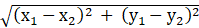So, r == [(2-0)2 + (-3-½)2]

= [(2)2 + (-7/2)2]

= [4 + 49/4]

= [65/4]

= /2

We know that the equation of the circle with centre (p, q) and having radius ‘r’ is given by: (x – p)2 + (y – q)2 = r2

Now by substituting the values in the above equation, we get

(x – 0)2 + (y – ½)2 = [/2]2

x2 + y2 – y + ¼ = 65/4

4x2 + 4y2 – 4y + 1 = 65

∴The equation of the circle is 4x2 + 4y2 – 4y – 64 = 0 or x2 + y2 – y – 16 = 0

2. Find the equation of the circle the end points of whose diameter are the centres of the circles x2 + y2 + 6x – 14y – 1 = 0 and x2 + y2 – 4x + 10y – 2 = 0.

Solution:

Given:

x2 + y2 + 6x – 14y – 1 = 0…. (1)

So the centre = [(-6/2), -(-14/2)]

= [-3, 7]

x2 + y2 – 4x + 10y – 2 = 0… (2)

So the centre = [-(-4/2), (-10/2)]

= [2, -5]

We know that the equation of the circle is given by,

(x – x1) (x – x2) + (y – y1) (y – y2) = 0

(x + 3) (x – 2) + (y – 7) (y + 5) = 0

Upon simplification we get

x2 + 3x – 2x – 6 + y2 – 7y + 5y – 35 = 0

x2 + y2 + x – 2y – 41 = 0

∴The equation of the circle is x2 + y2 + x – 2y – 41 = 0

3. The sides of a squares are x = 6, x = 9, y = 3 and y = 6. Find the equation of a circle drawn on the diagonal of the square as its diameter.

Solution:

Given:

The sides of a squares are x = 6, x = 9, y = 3 and y = 6.

Let us assume A, B, C, D be the vertices of the square. On solving the lines, we get the coordinates as: A = (6, 3)

B = (9, 3)

C = (9, 6)

D = (6, 6)

We know that the equation of the circle with diagonal AC is given by

(x – x1) (x – x2) + (y – y1) (y – y2) = 0

(x – 6) (x – 9) + (4 – 3) (4 – 6) = 0

Upon simplifying, we get

x2 – 6x – 9x + 54 + y2 – 3y – 6y + 18 = 0

x2 + y2 – 15x – 9y + 72 = 0

We know that the equation of the circle with diagonal BD as diameter is given by

(x – x1) (x – x2) + (y – y1) (y – y2) = 0

(x – 9) (x – 6) + (y – 3) (y – 6) = 0

Upon simplifying, we get

x2 – 9x – 6x + 54 + y2 – 3y – 6y + 18 = 0

x2 + y2 – 15x – 9y + 72 = 0

∴The equation of the circle is x2 + y2 – 15x – 9y + 72 = 0

4. Find the equation of the circle circumscribing the rectangle whose sides are x – 3y = 4, 3x + y = 22, x – 3y = 14 and 3x + y = 62.

Solution:

Given:

The sides x – 3y = 4 …. (1)

3x + y = 22 … (2)

x – 3y = 14 …. (3)

3x + y = 62 … (4)

Let us assume A, B, C, D be the vertices of the square. On solving the lines, we get the coordinates as: A = (7, 1)

B = (8, – 2)

C = (20, 2)

D = (19, 5)

We know that the equation of the circle with diagonal AC as diameter is given by

(x – x1) (x – x2) + (y – y1) (y – y2) = 0

(x – 7) (x – 20) + (y – 1) (y – 2) = 0

Upon simplification we get

x2 + y2 – 27x – 3y + 142 = 0

∴The equation of the circle is x2 + y2 – 27x – 3y + 142 = 0

5. Find the equation of the circle passing through the origin and the points where the line 3x + 4y = 12 meets the axes of coordinates.

Solution:

Given:

The line 3x + 4y = 12

The value of x is 0 on meeting the y – axis. So,

3(0) + 4y = 12

4y = 12

y = 3

The point is A(0, 3)

The value of y is 0 on meeting the x – axis. So,

3x + 4(0) = 12

3x = 12

x = 4

The point is B(4, 0)

Since the circle passes through origin and A and B

So, AB is the diameter

We know that the equation of the circle with AB as diameter is given by

(x – x1) (x – x2) + (y – y1) (y – y2) = 0

(x – 0) (x – 4) + (y – 3) (y – 0) = 0

x2 + y2 – 4x – 3y = 0

∴The equation of the circle is x2 + y2 – 4x – 3y = 0

6. Find the equation of the circle which passes through the origin and cuts off intercepts a and b respectively from x and y – axes.

Solution:

Since the circle has intercept ‘a’ from x – axis, the circle must pass through (a, 0) and (-a, 0) as it already passes through the origin.

Since the circle has intercept ‘b’ from x – axis, the circle must pass through (0, b) and (0, -b) as it already passes through the origin.

Let us assume the circle passing through the points A(a,0) and B(0,b).

We know that the equation of the circle with AB as diameter is given by

(x – x1) (x – x2) + (y – y1) (y – y2) = 0

(x – a) (x – 0) + (y – 0) (y – b) = 0

x2 + y2 + ax + by = 0 or x2 + y2 – ax – by = 0

∴The equation of the circle is x2 + y2 + ax + by = 0 or x2 + y2 – ax – by = 0

### Also, access exercises of RD Sharma Solutions for Class 11 Maths Chapter 24 – The Circle

Exercise 24.1 Solutions

Exercise 24.2 Solutions

Exercise 24.3 Solutions

## Frequently Asked Questions on RD Sharma Solutions for Class 11 Maths Chapter 24

### What is the importance of using the RD Sharma Solutions for Class 11 Maths Chapter 24?

The importance of using the RD Sharma Solutions for Class 11 Maths Chapter 24 are:
1. Subject experts have created the solutions carefully according to the latest CBSE guidelines.
2. The solutions PDF help students during their revisions.
3. Provide accurate answers to all the textbook questions present in the textbook.
4. All the fundamentals and complex topics are explained in simple steps.

### Is the RD Sharma Solutions for Class 11 Maths Chapter 24 from BYJU’S enough for students to prepare for their exams?

The RD Sharma Solutions for Class 11 Maths Chapter 24 from BYJU’S is created by subject experts having vast experience in the subject. By practicing the RD Sharma Solutions provided at BYJU’S website on a regular basis students can also improve their confidence in attempting various competitive exams. All the questions are covered and solved in simple steps for easy understanding of topics to help students grasp the concepts at ease.

### What are the benefits of referring to the RD Sharma Solutions for Class 11 Maths Chapter 24?

Every student has to take up exams as they are the basis of assessing how knowledgeable and informational a student is. The RD Sharma Solutions for Class 11 Maths Chapter 24 provides precise and detailed answers to the textbook questions. All the basic concepts are explained in a simple and comprehensive manner to aid students to ace their annual exam. Students can also improve their problem solving and time management skills by regularly using these resources while preparing for exams.